# RD Sharma Solutions for Class 11 Maths Chapter 20 Geometric Progressions

## RD Sharma Solutions Class 11 Maths Chapter 20 – Download Free PDF Updated for 2021-22

RD Sharma Solutions for Class 11 Maths Chapter 20 – Geometric Progressions are provided here for students to score good marks in the board exams. A sequence of non-zero numbers is called a geometric progression if the ratio of a term and the term preceding it, is always a constant quantity.

This chapter solution is designed by our subject experts, to help understand the concepts and methods to solve problems in a shorter period and also to boost the confidence levels among students. RD Sharma Class 11 Maths Solutions are of immense help to students aiming to secure a good academic score in the board exams. To know more about the topics under this chapter, students can download the pdf of RD Sharma Solutions for Class 11 Maths Chapter 20 from the below-mentioned links.

Chapter 20 – Geometric Progressions contains six exercises and the RD Sharma Solutions present in this page provide solutions to the questions present in each exercise. Now, let us have a look at the concepts discussed in this chapter.

• The general term of a G.P.
• Selection of terms in G.P.
• Sum of the terms of a G.P.
• Sum of an infinite G.P.
• Properties of geometric progressions.
• Insertion of geometric means between two given numbers.
• Some important properties of arithmetic and geometric means.

## Download the pdf of RD Sharma Solutions for Class 11 Maths Chapter 20 – Geometric Progressions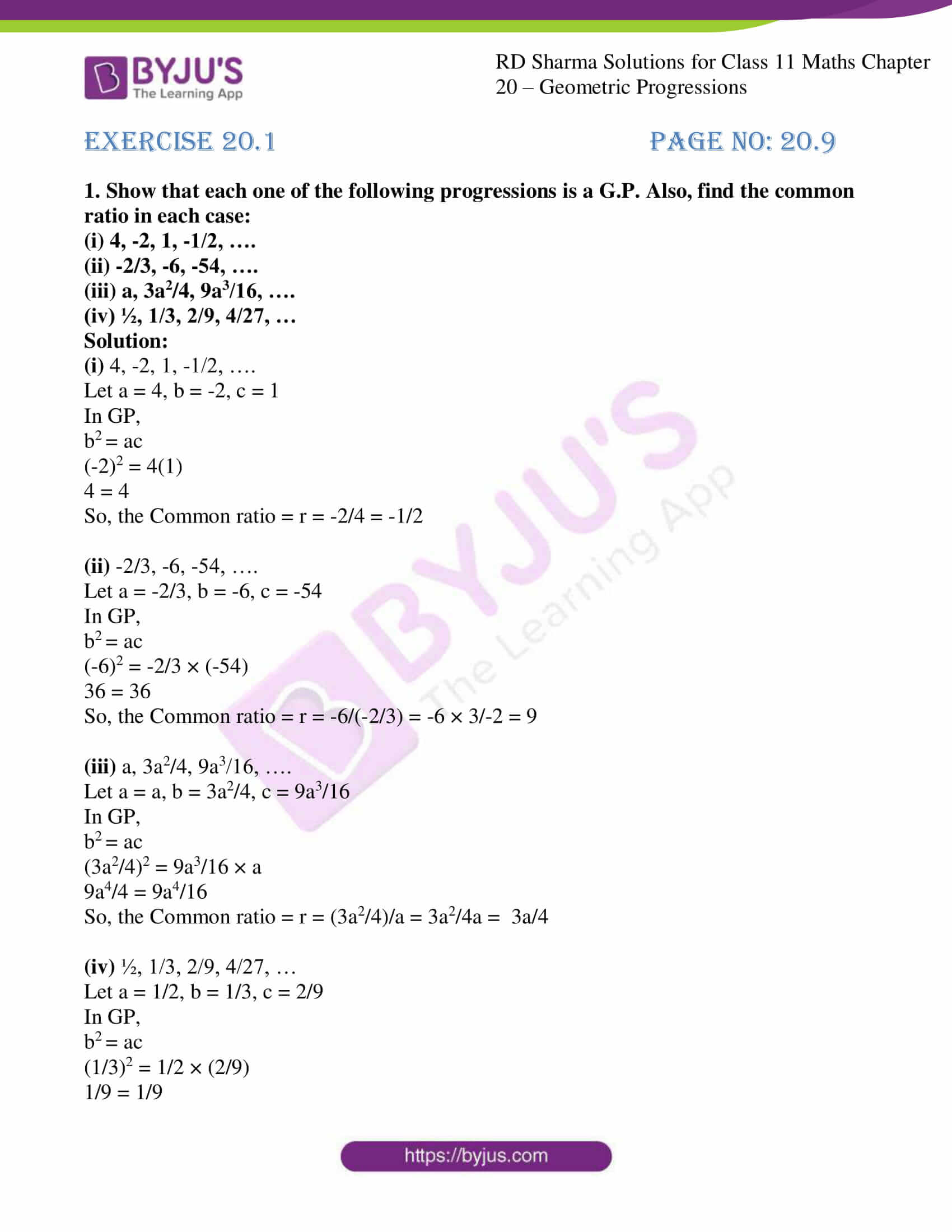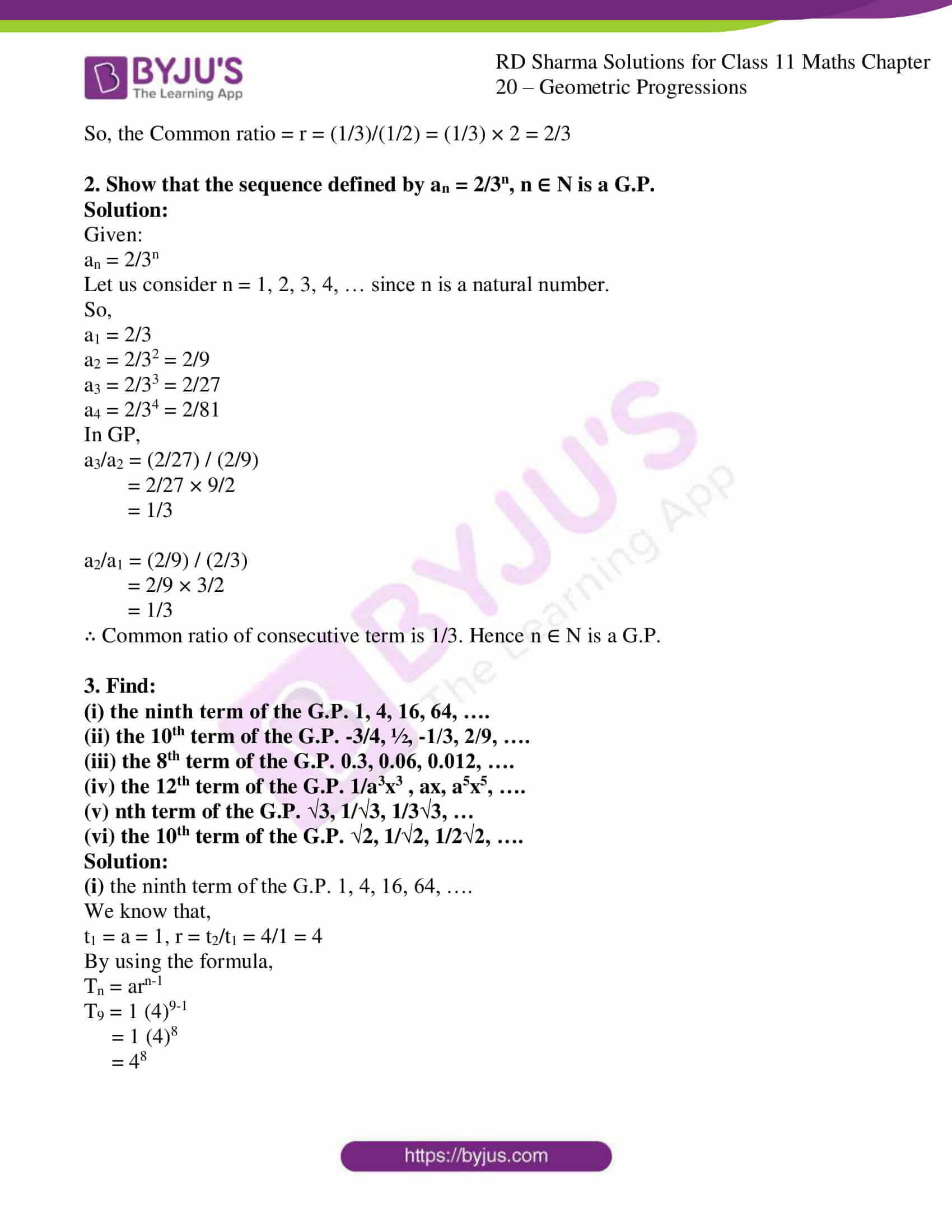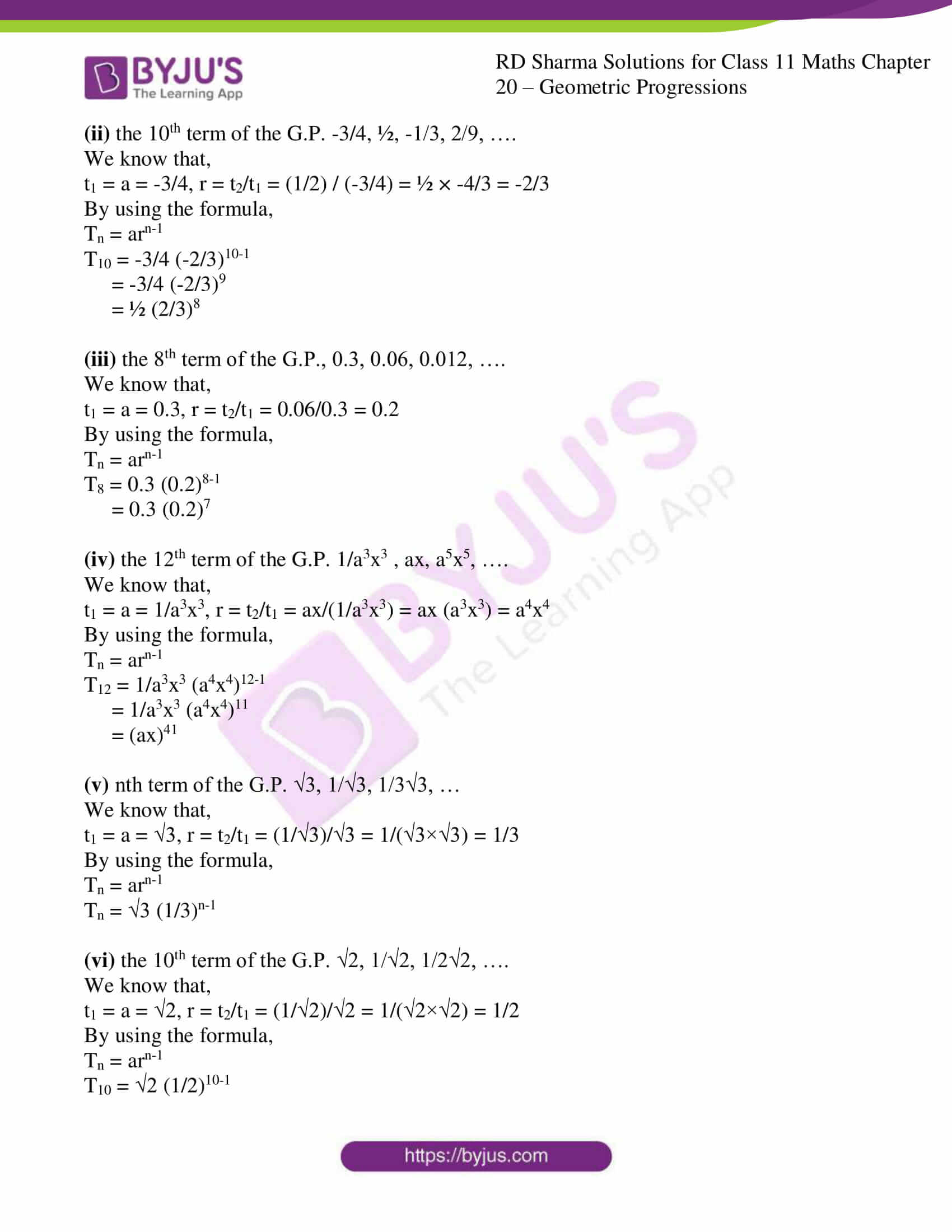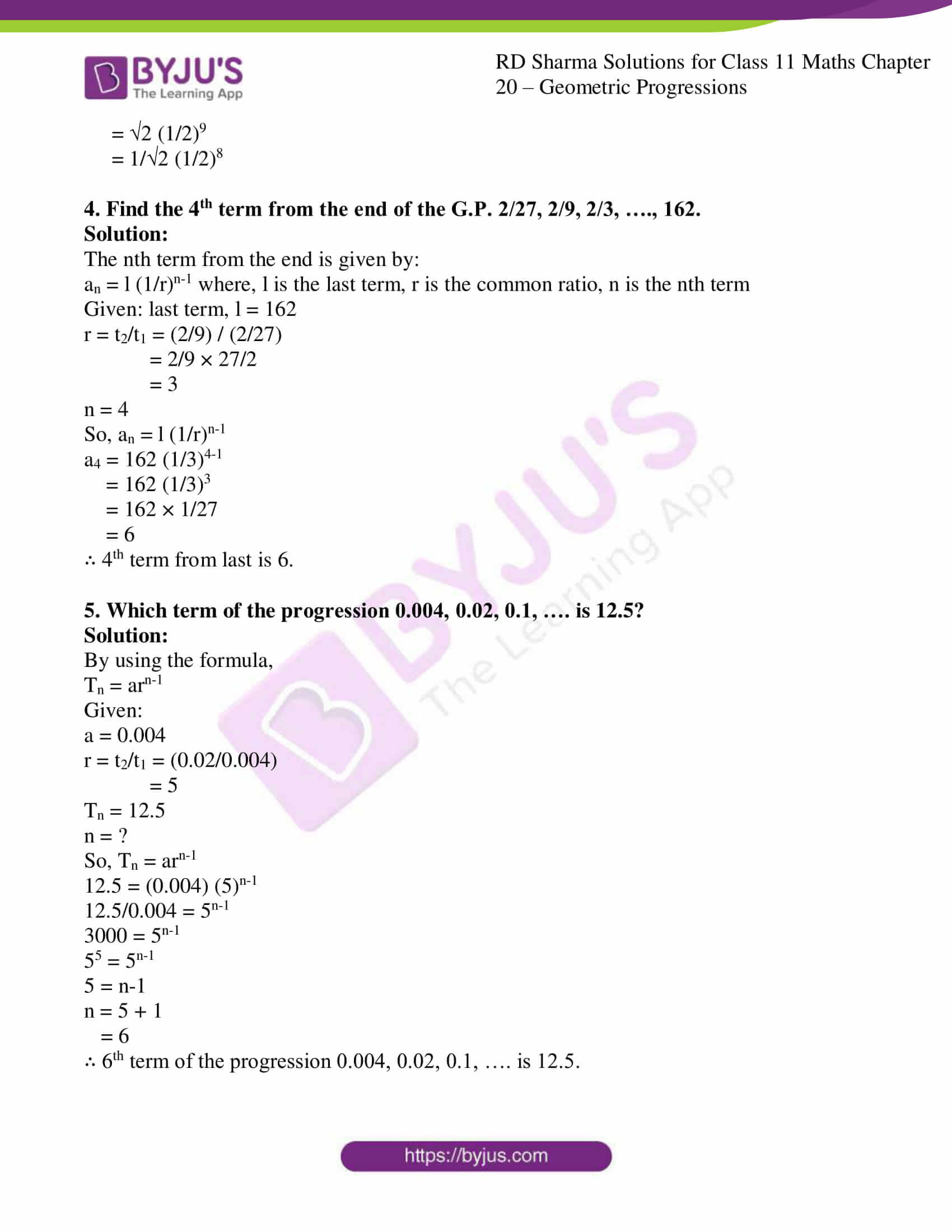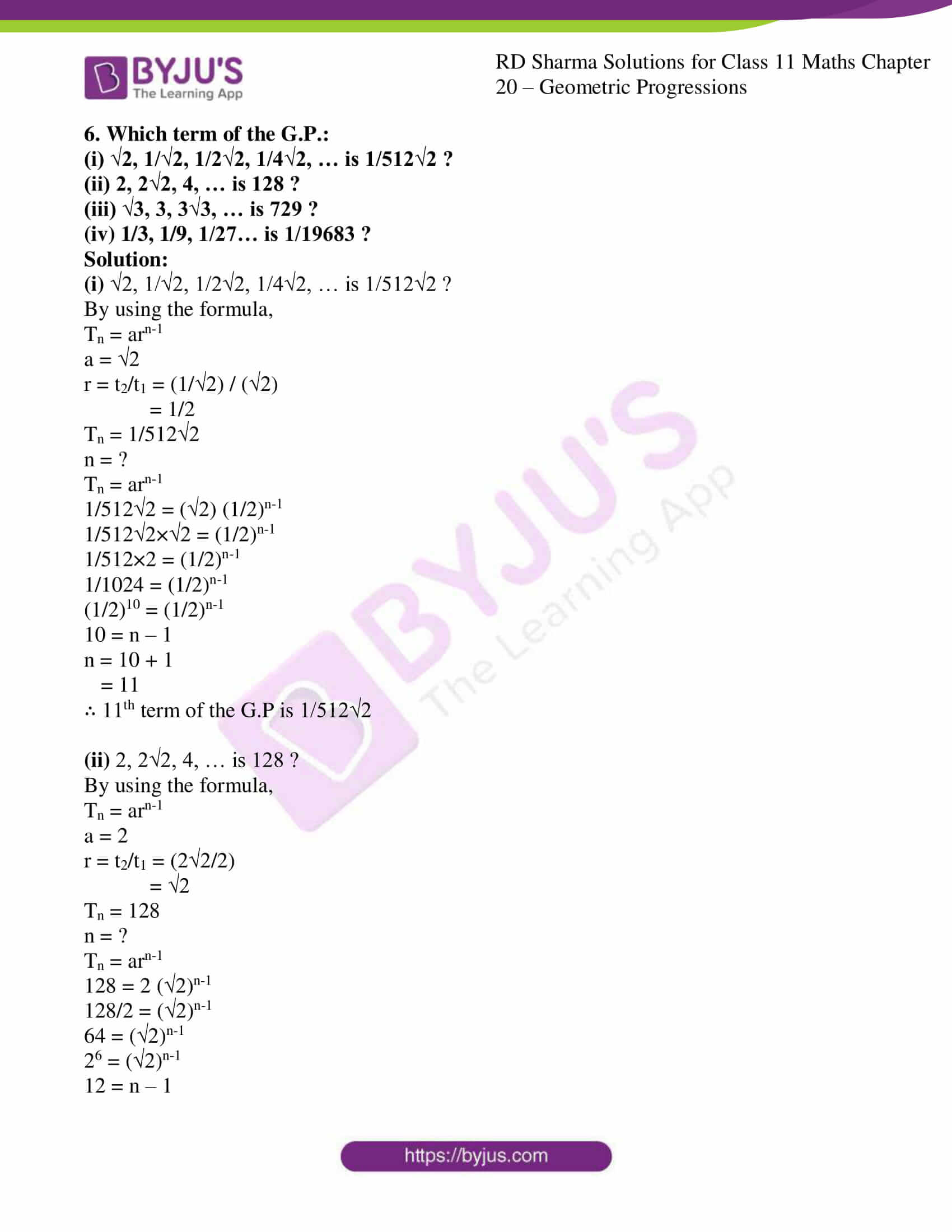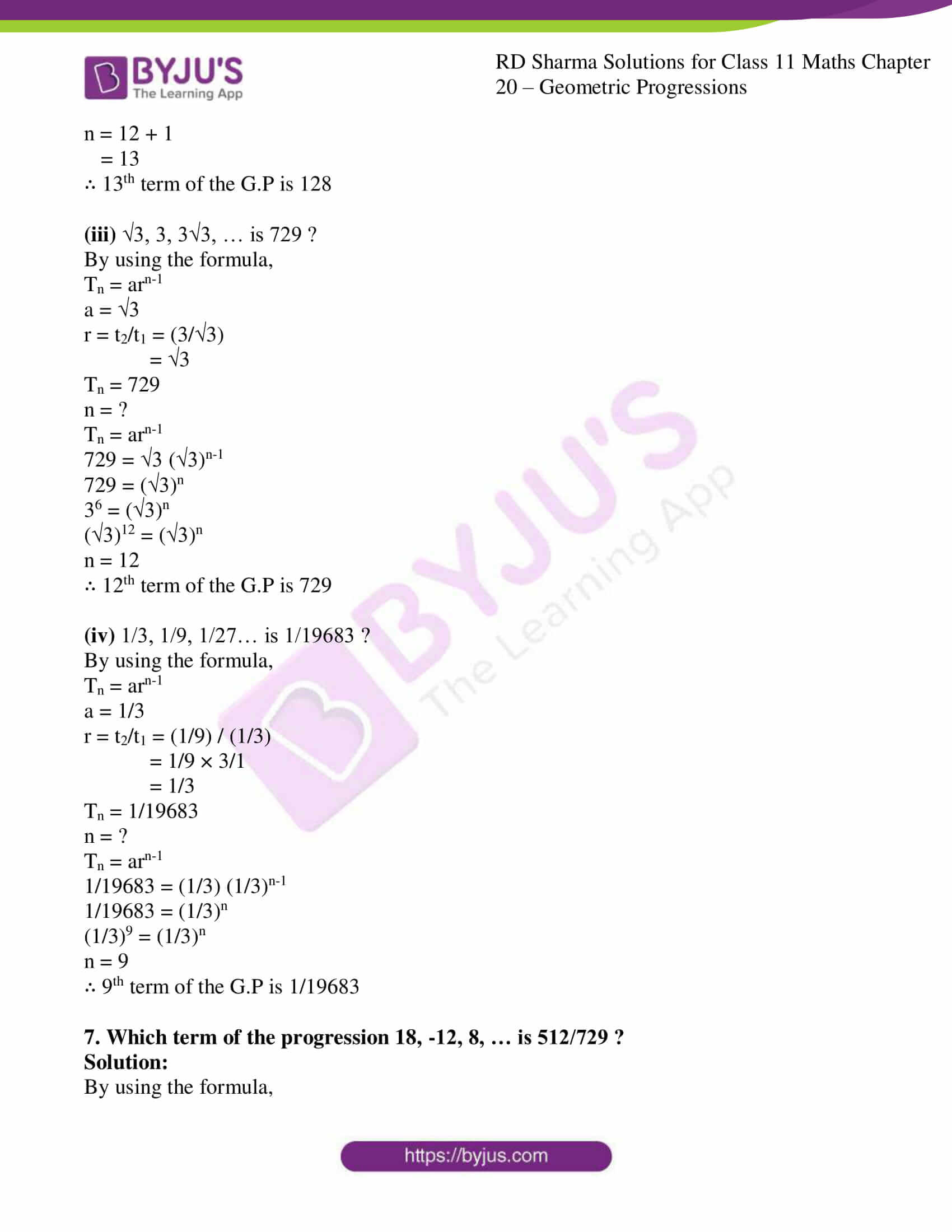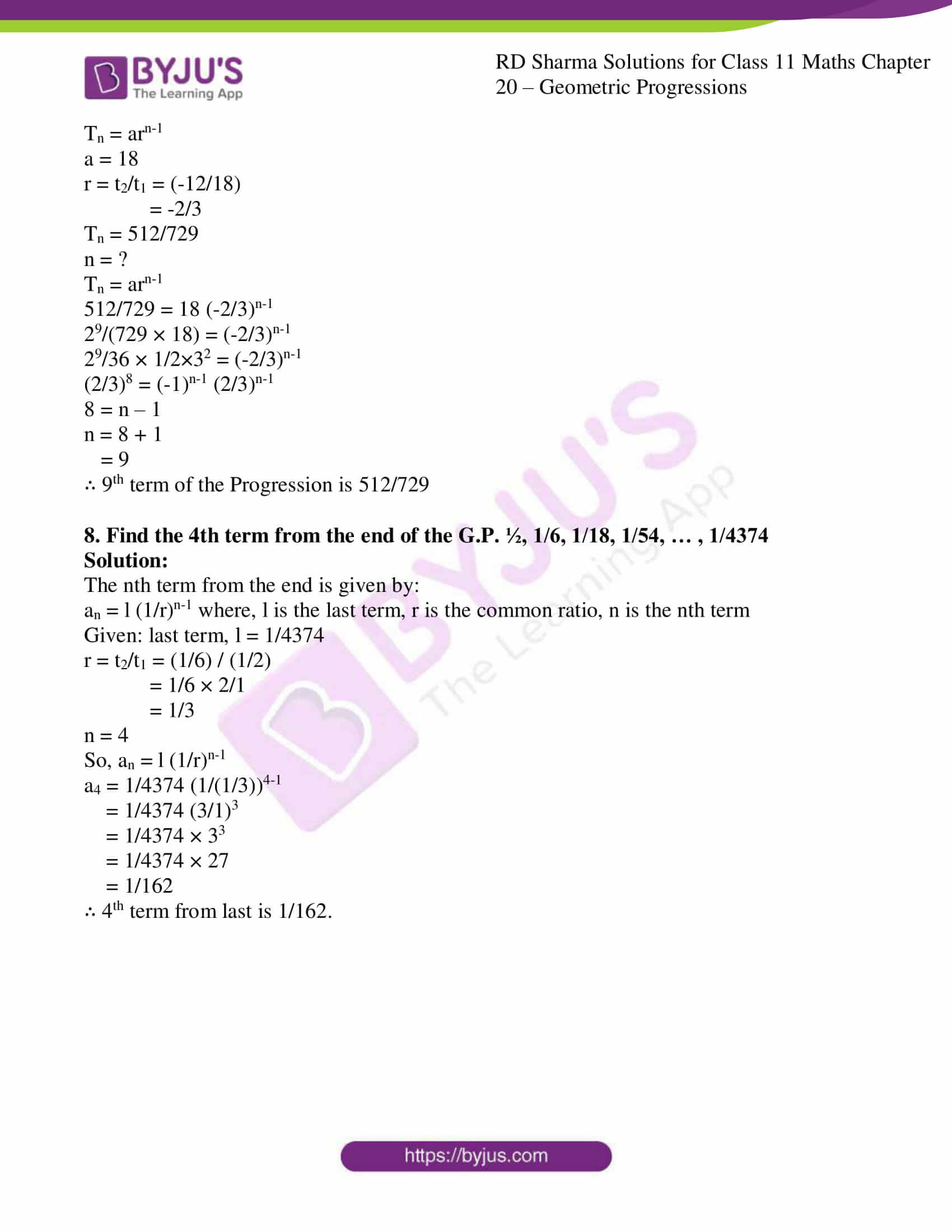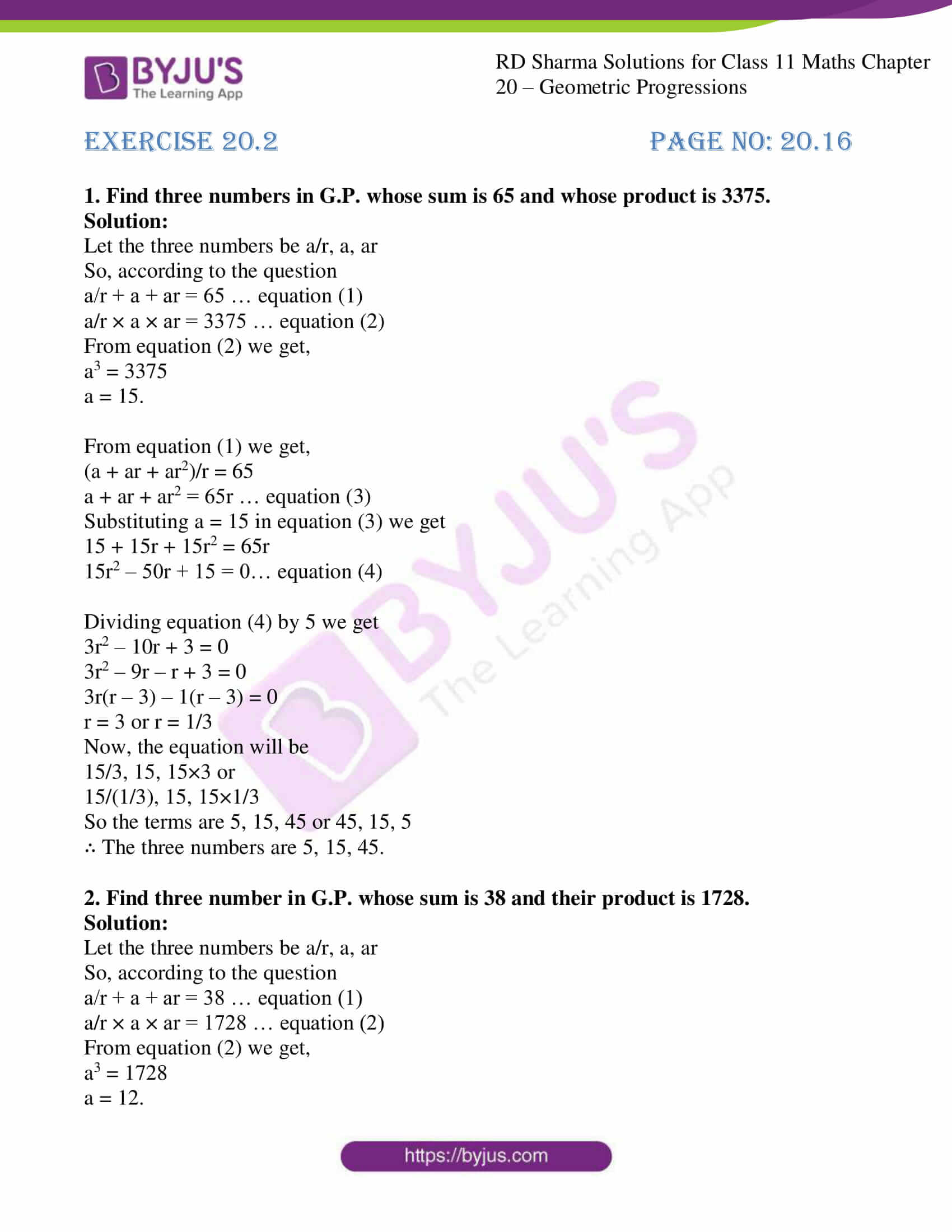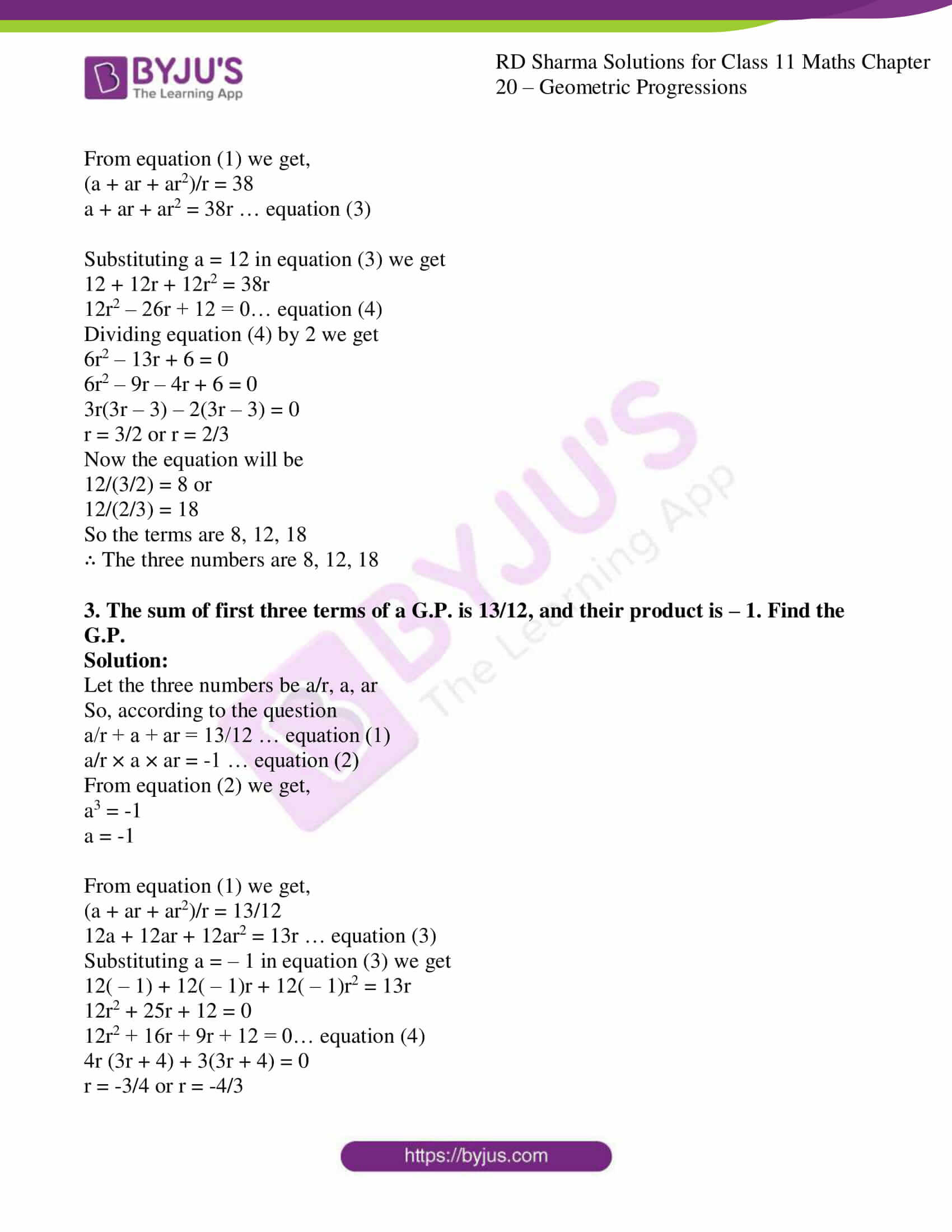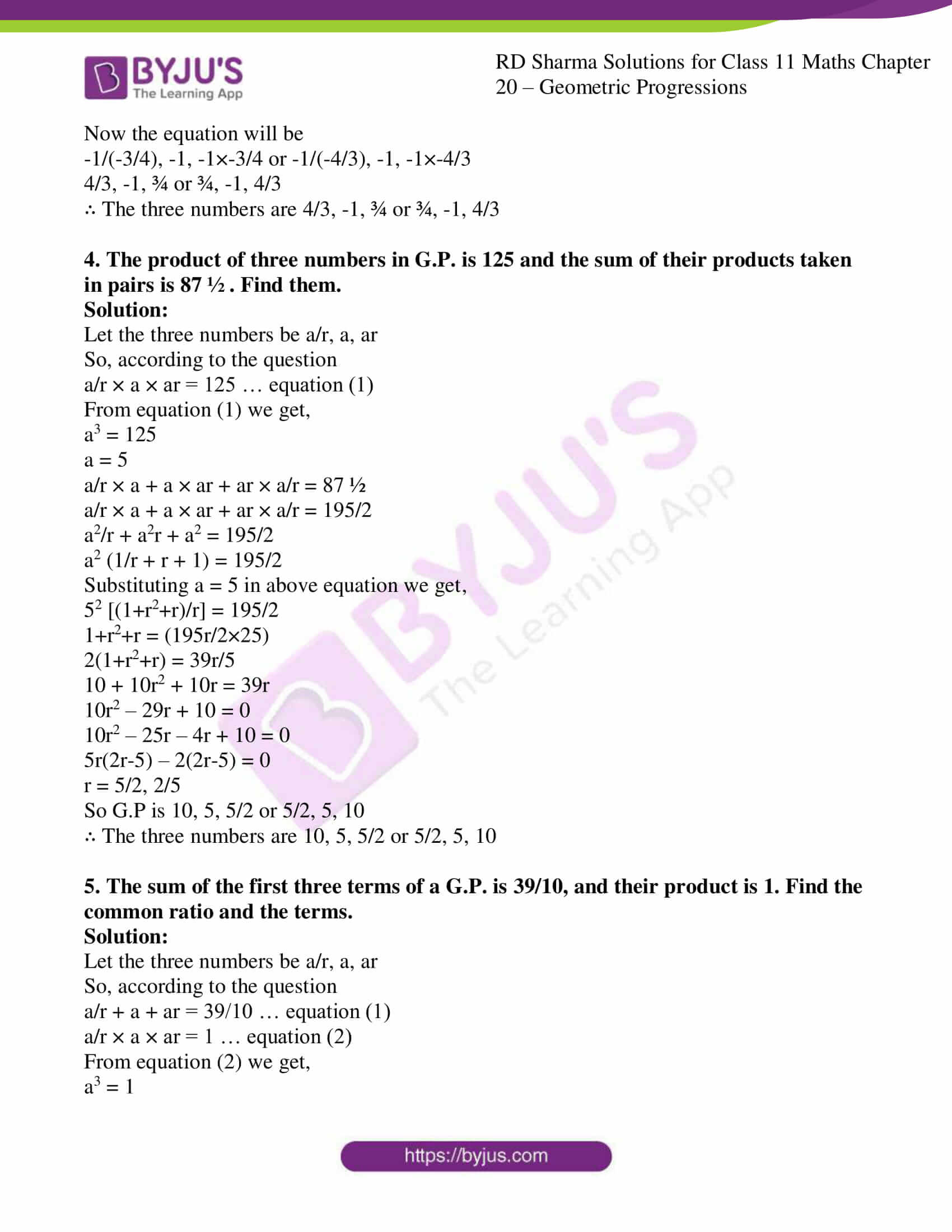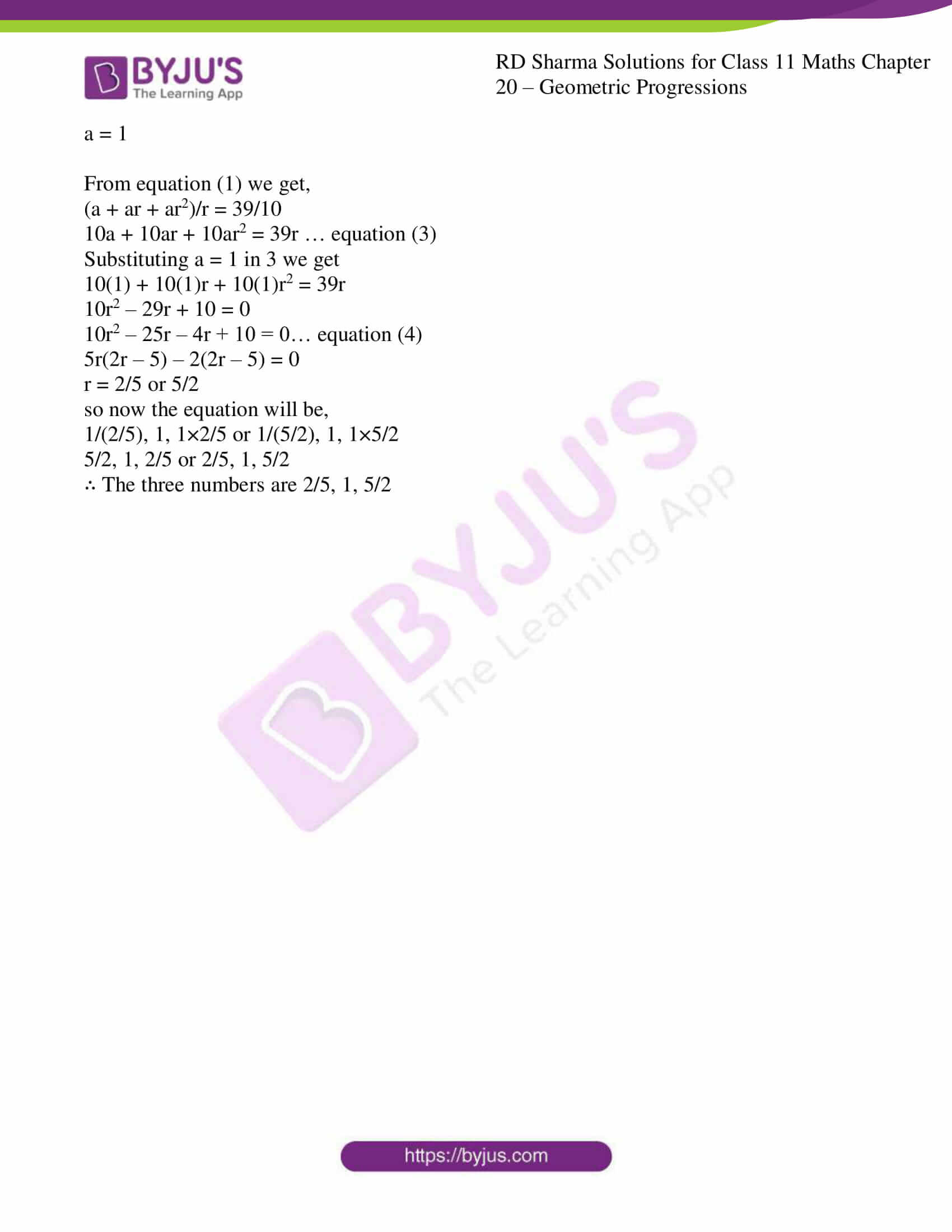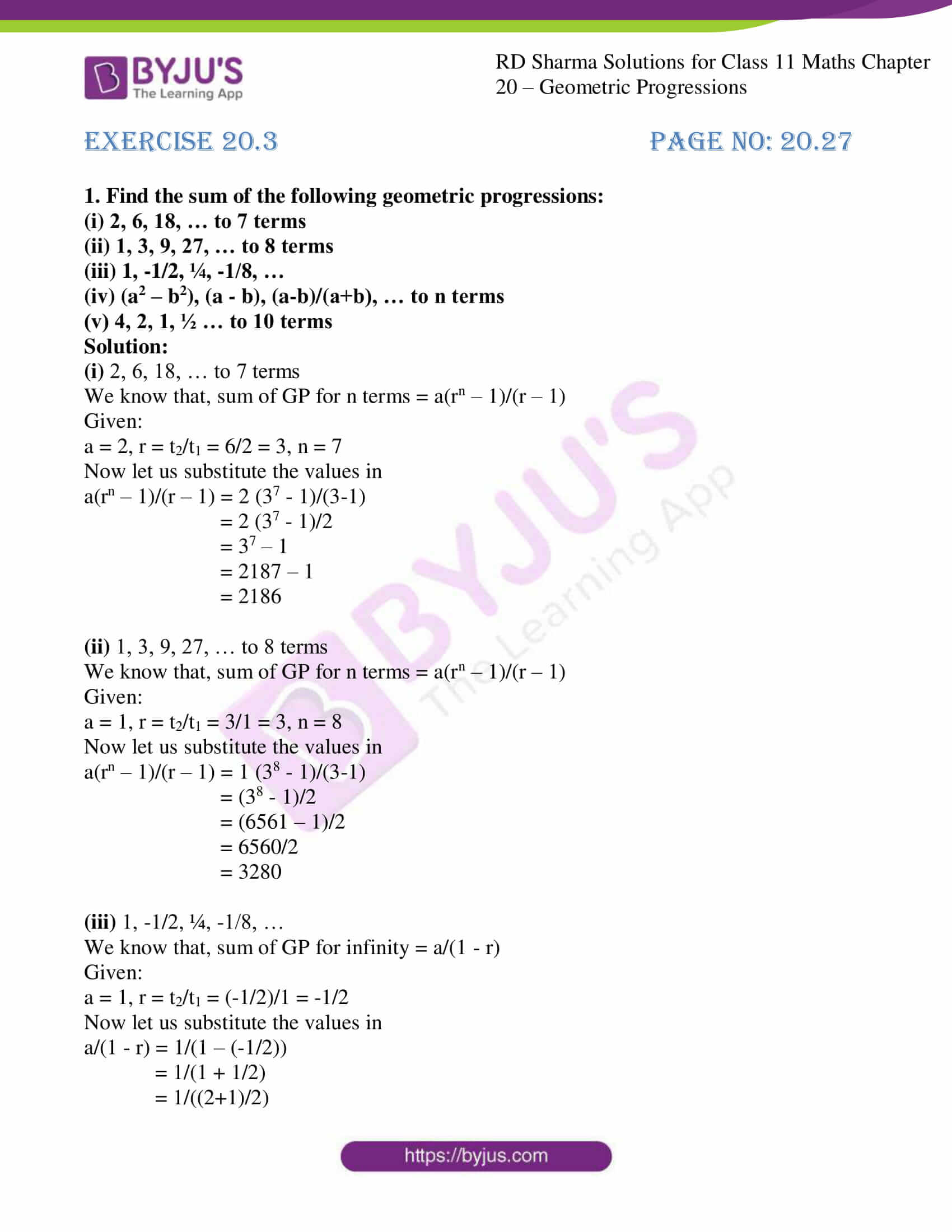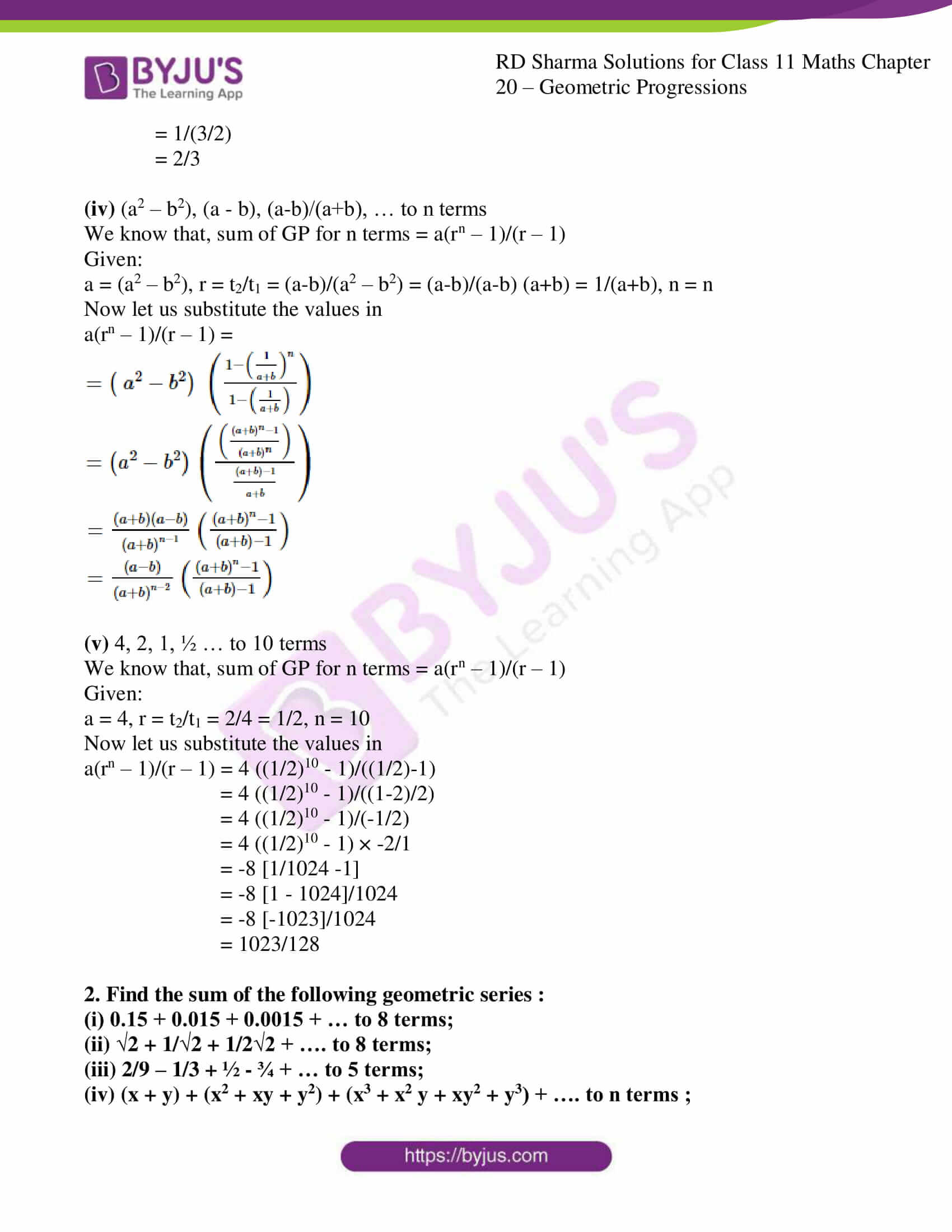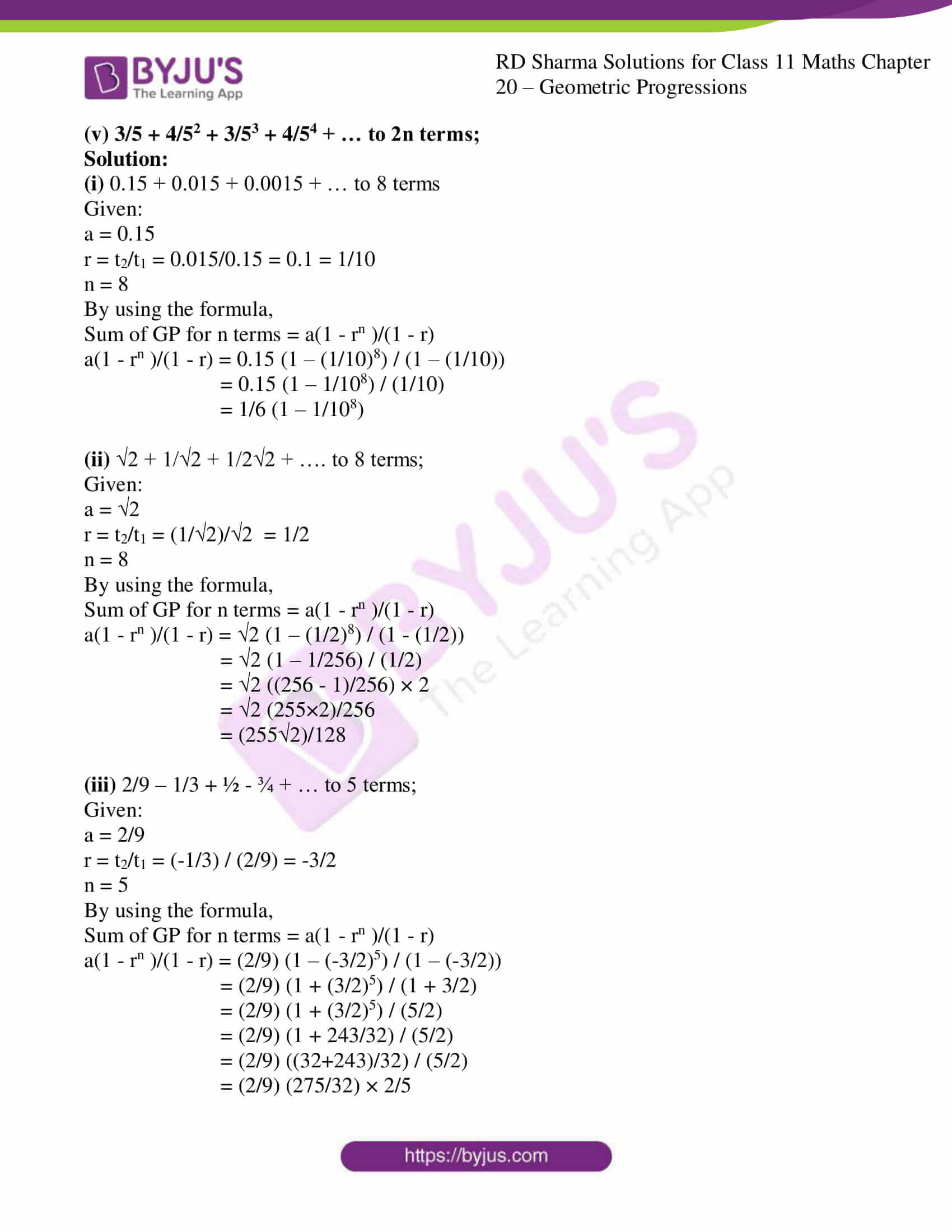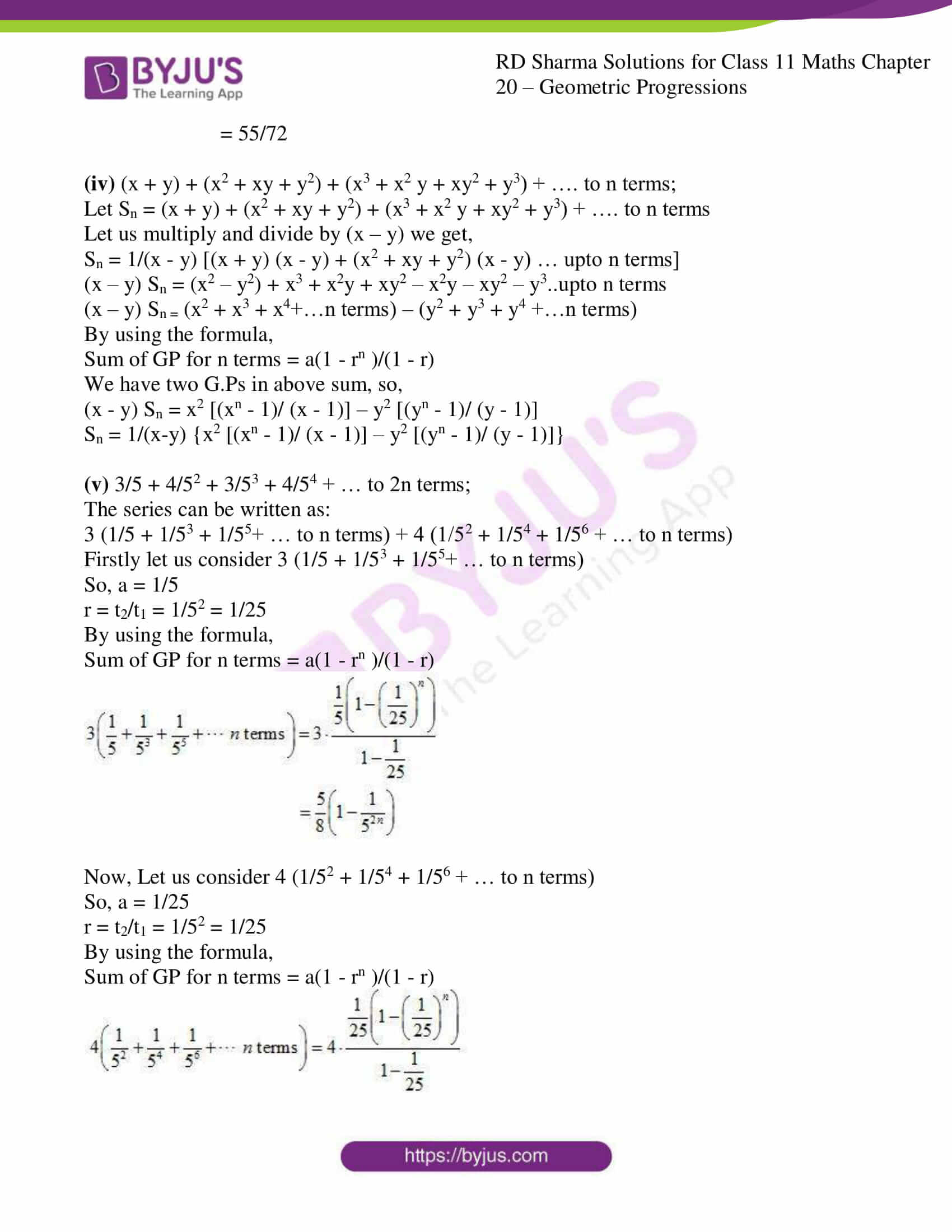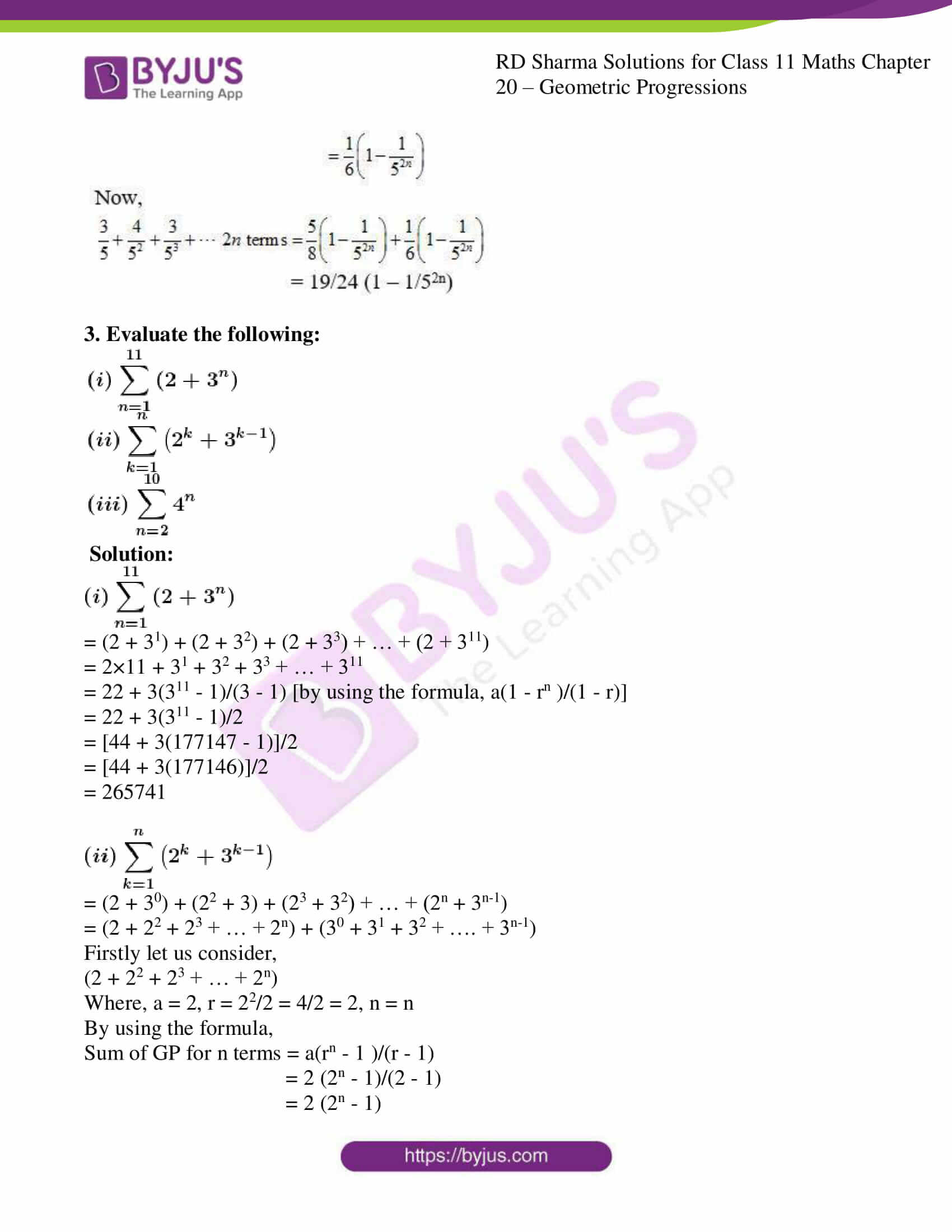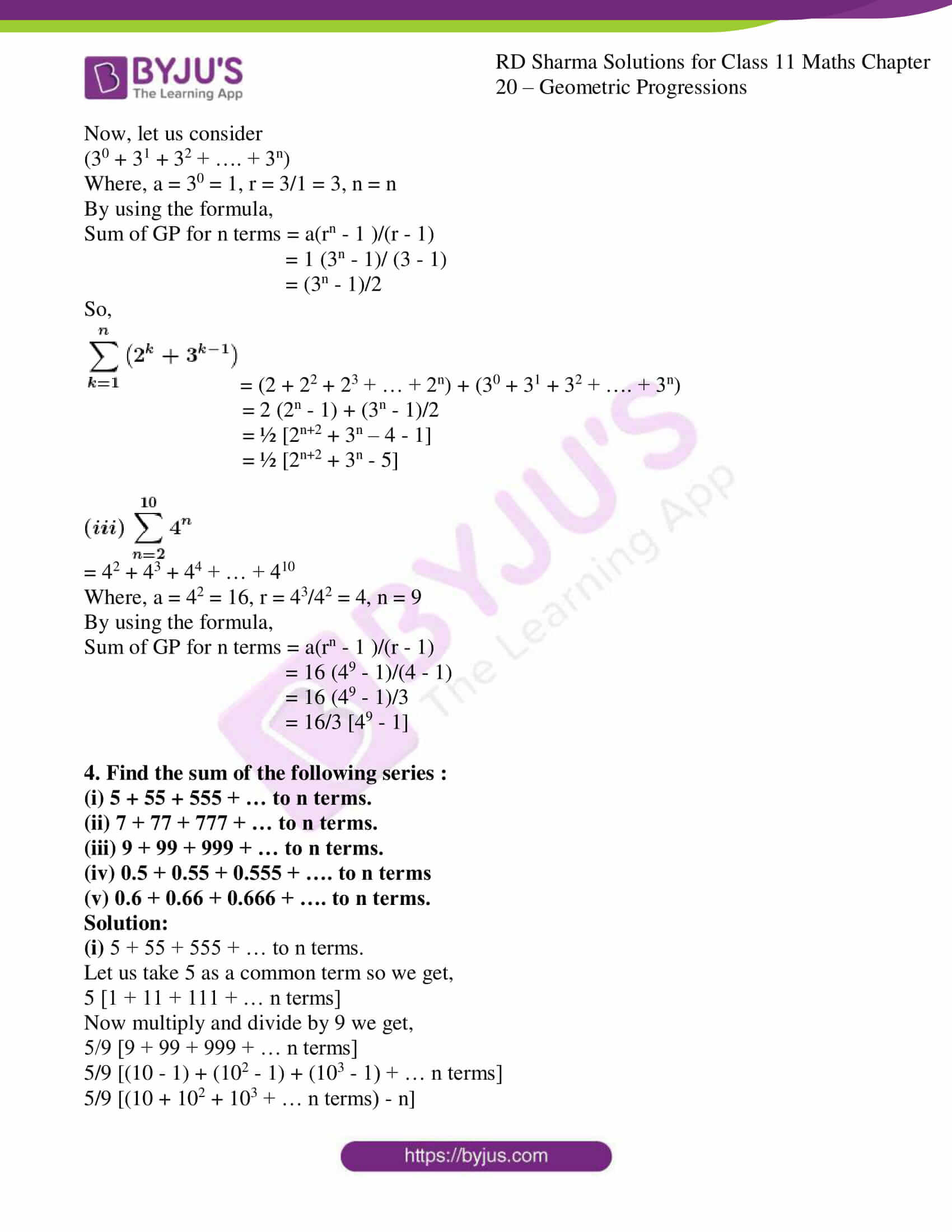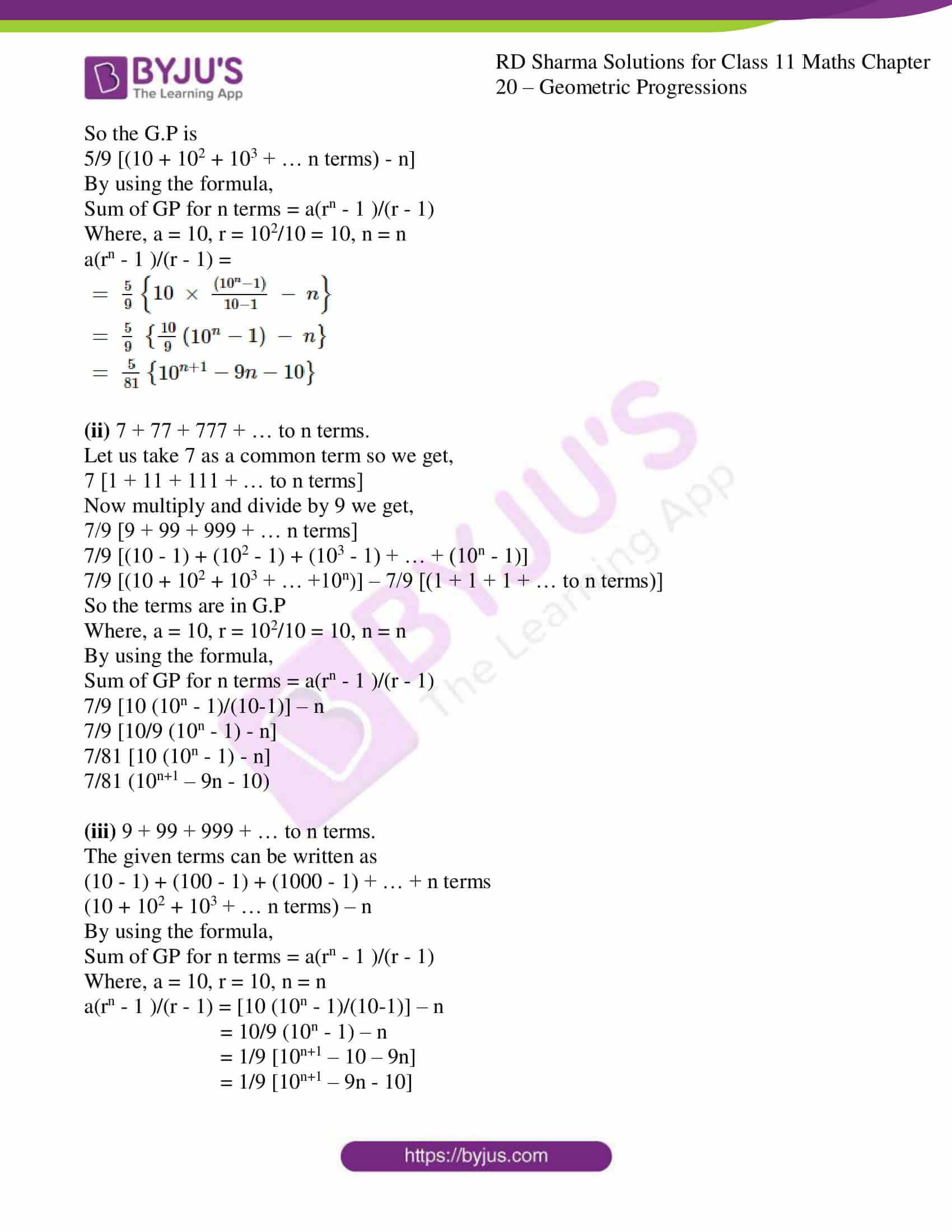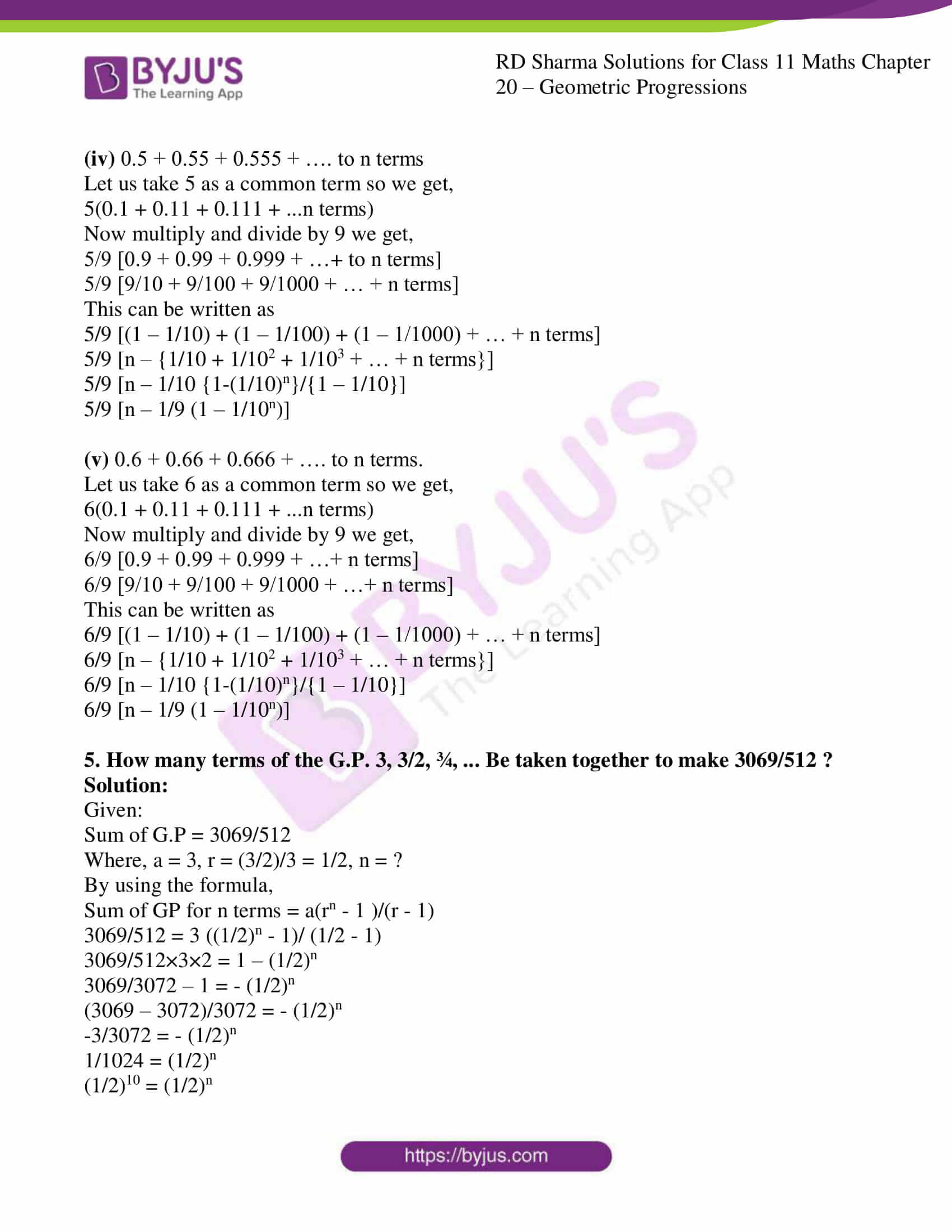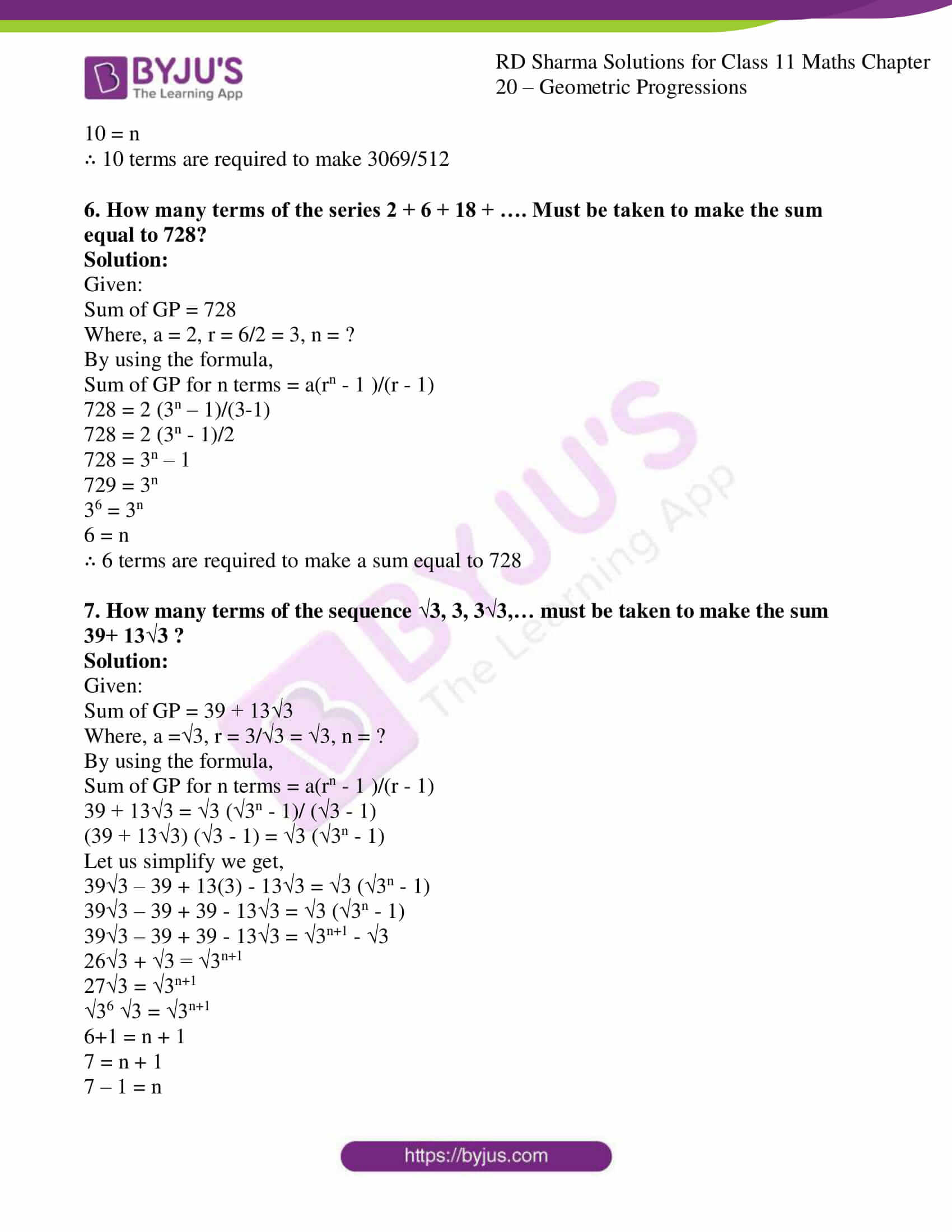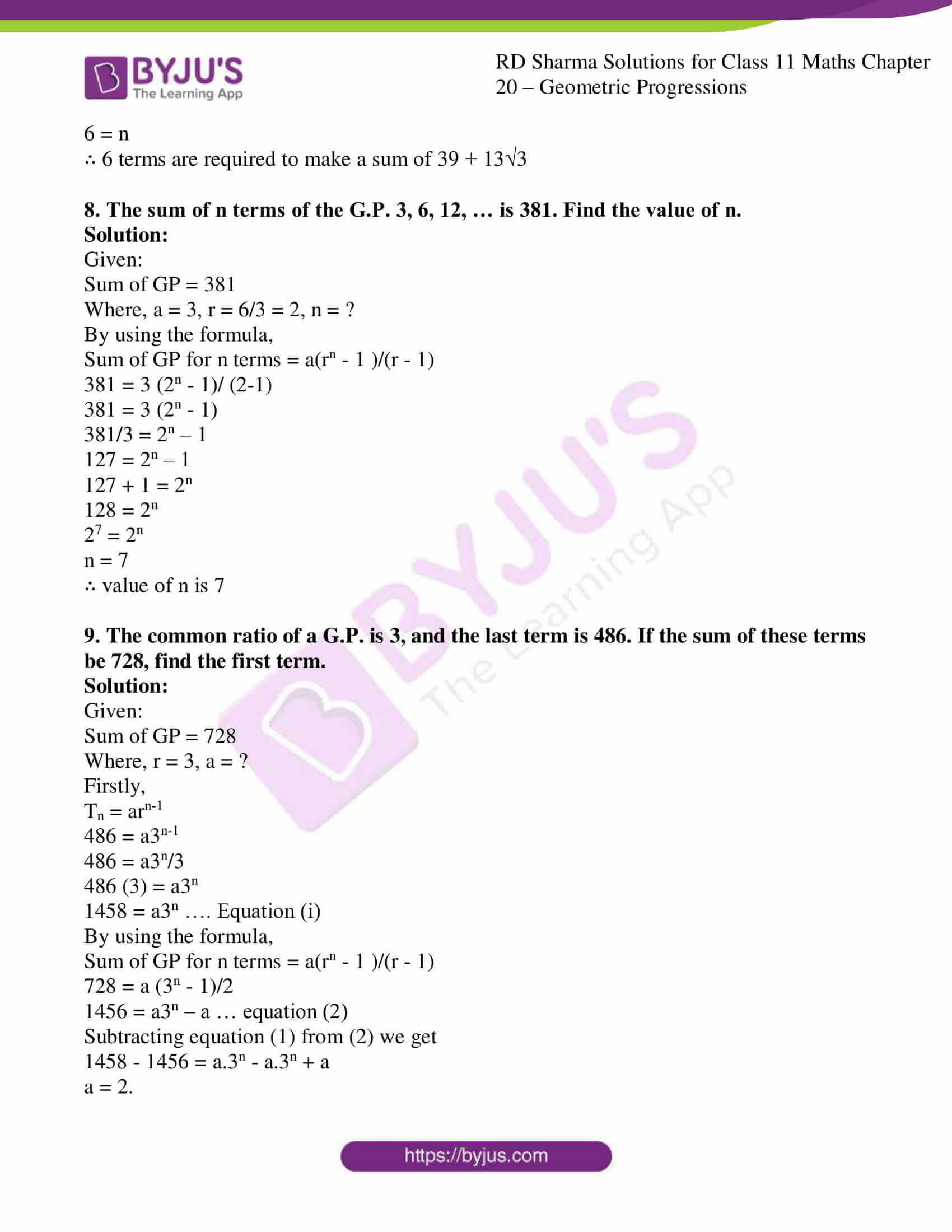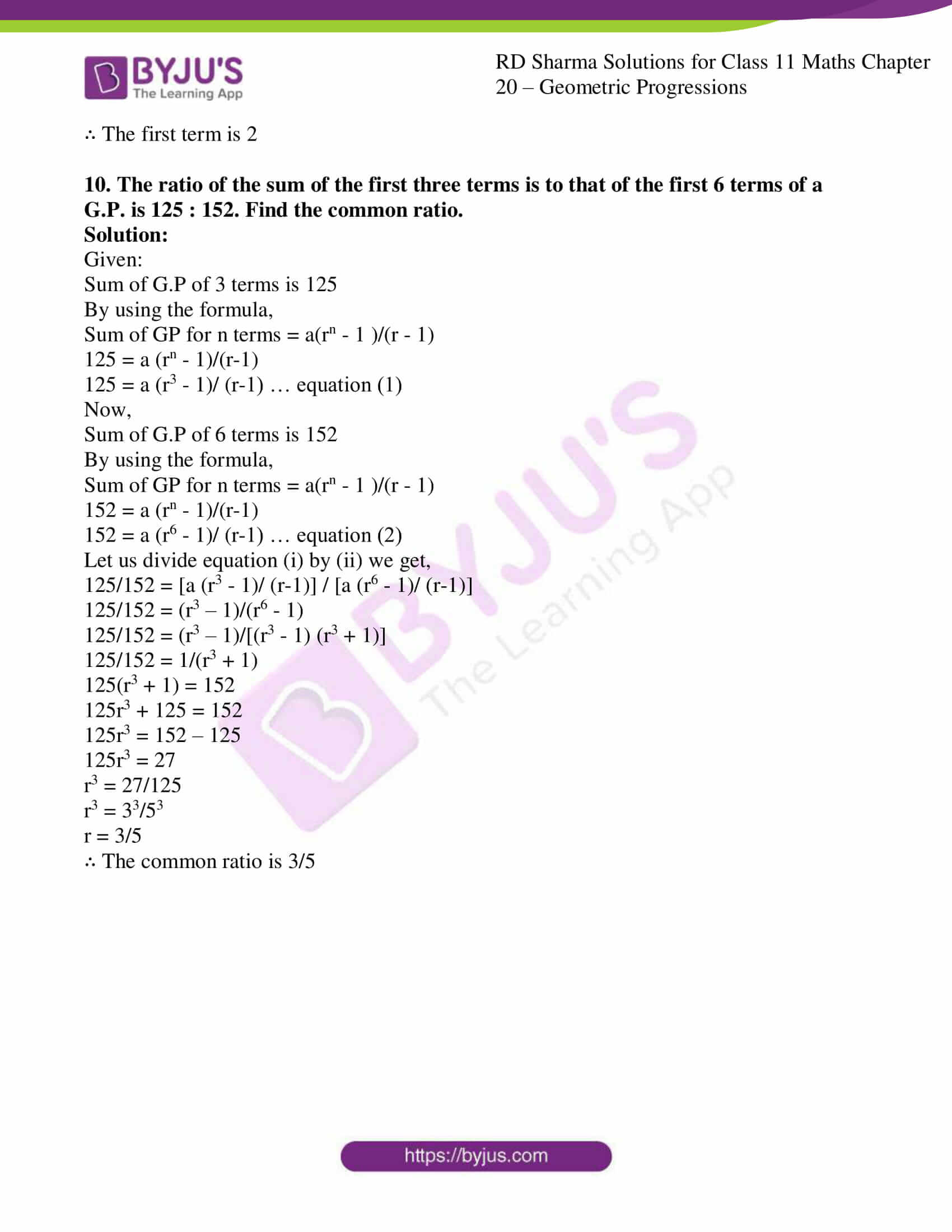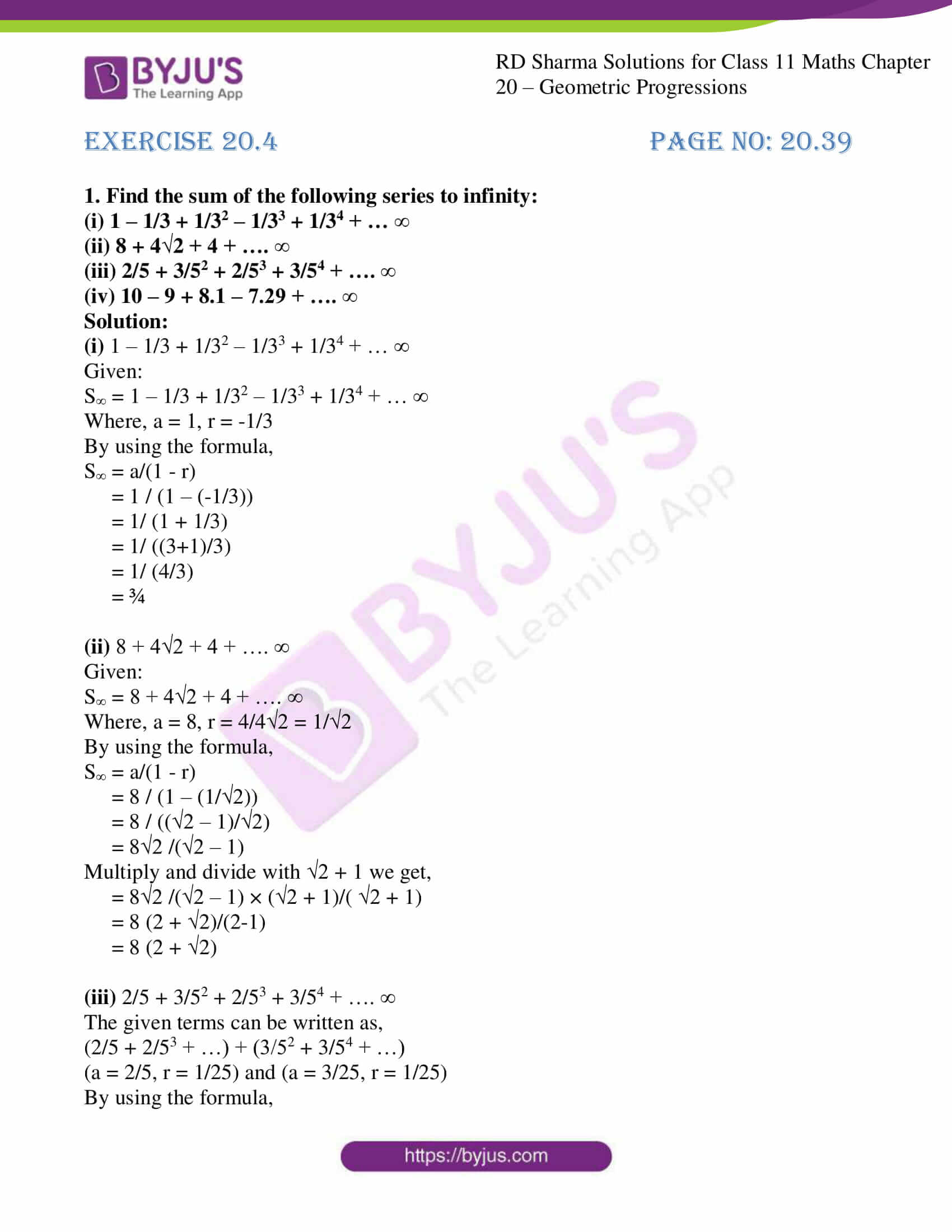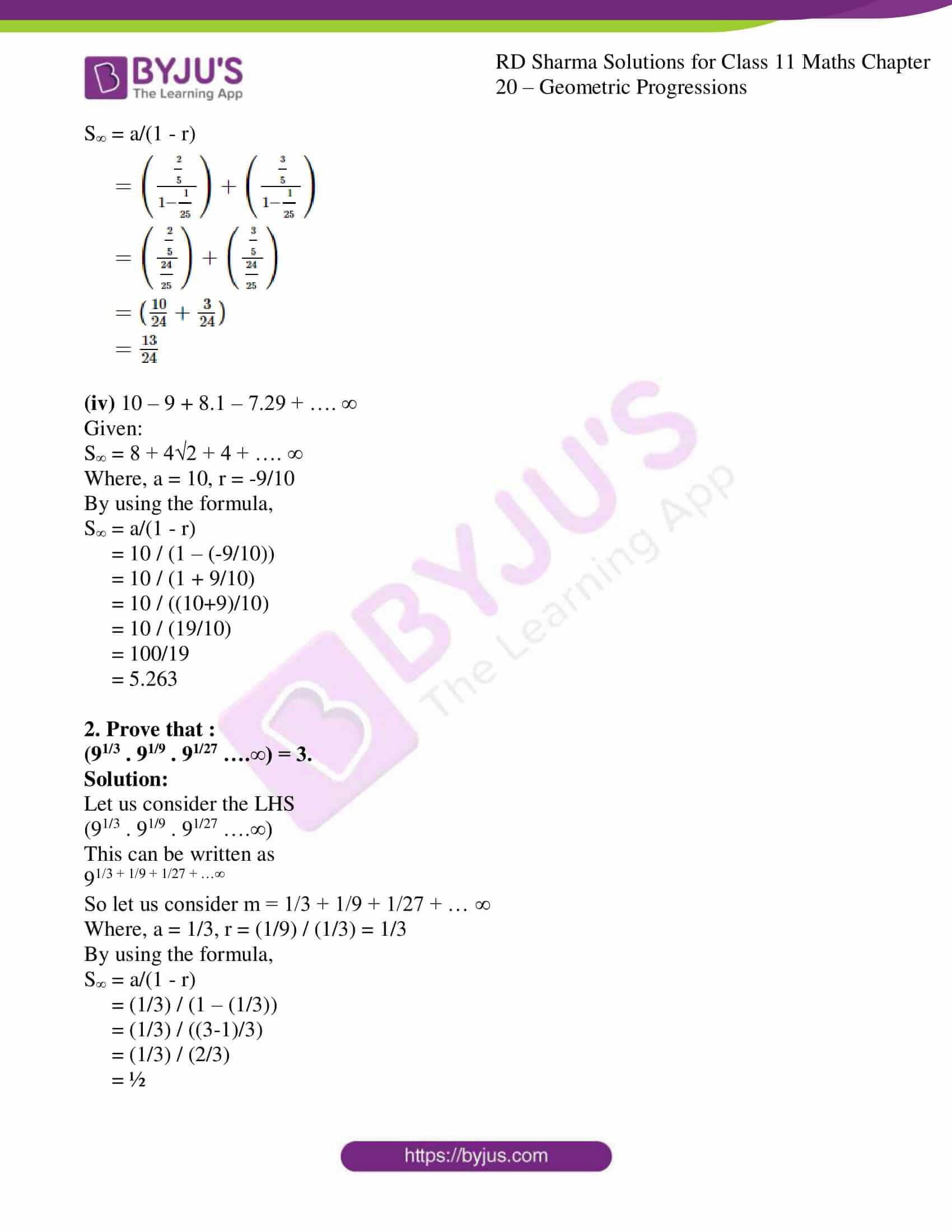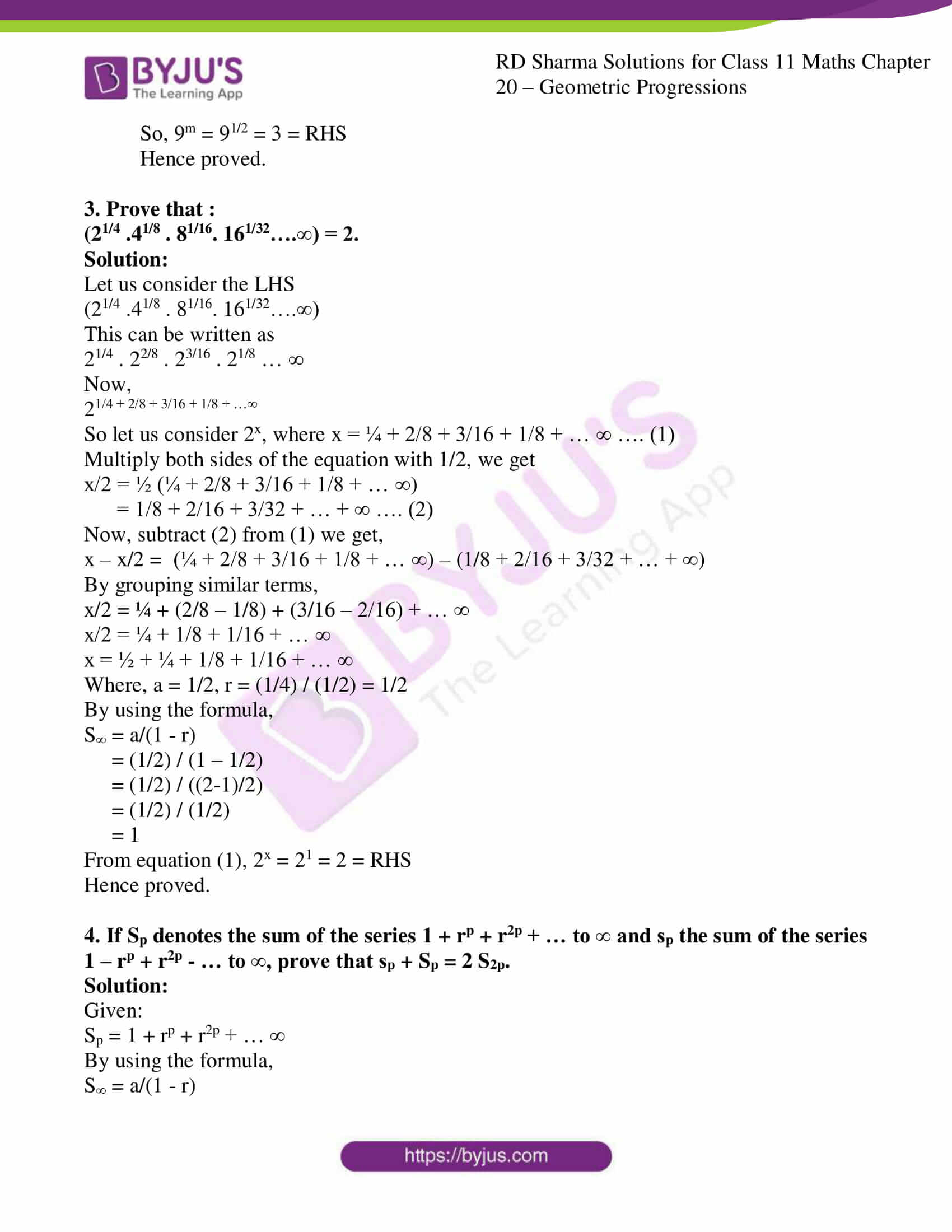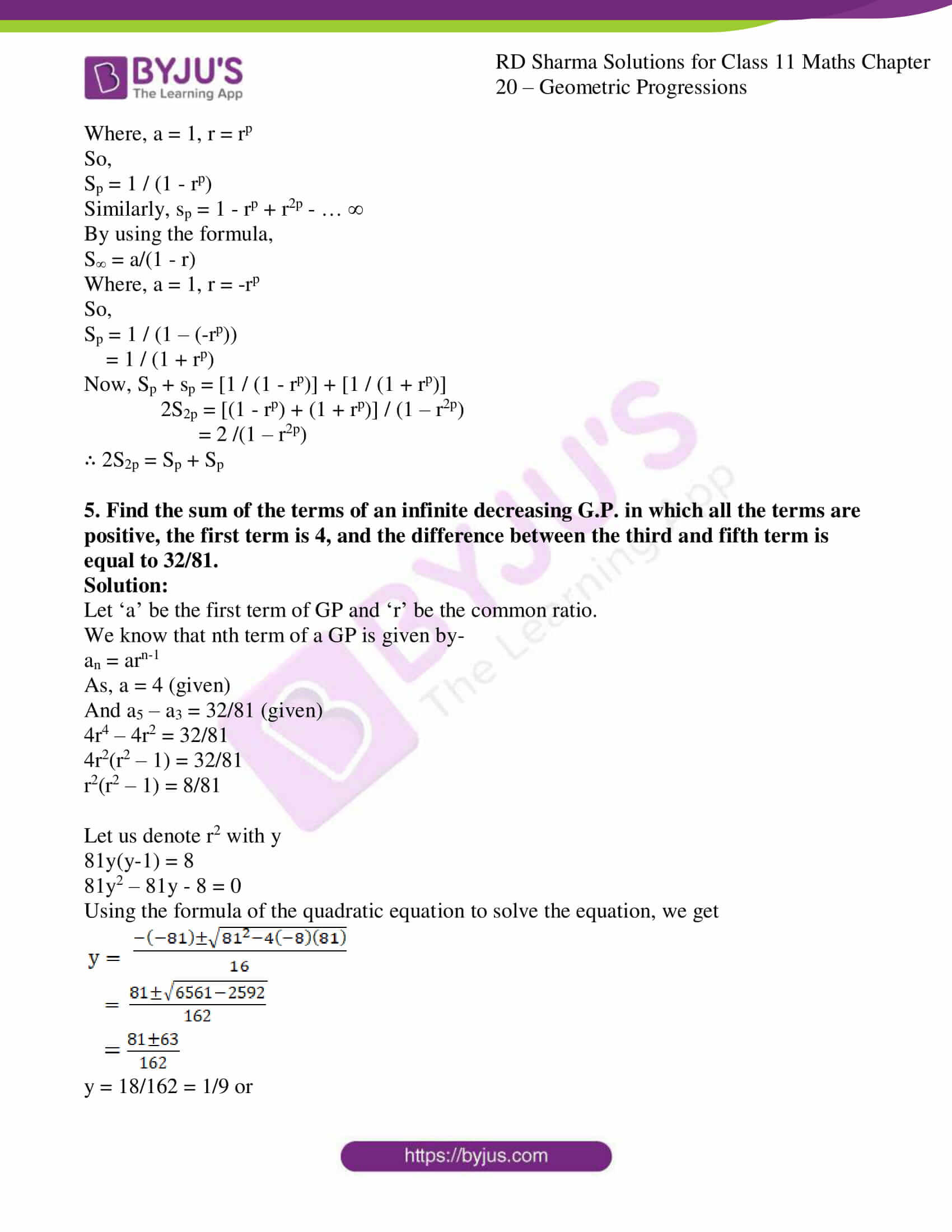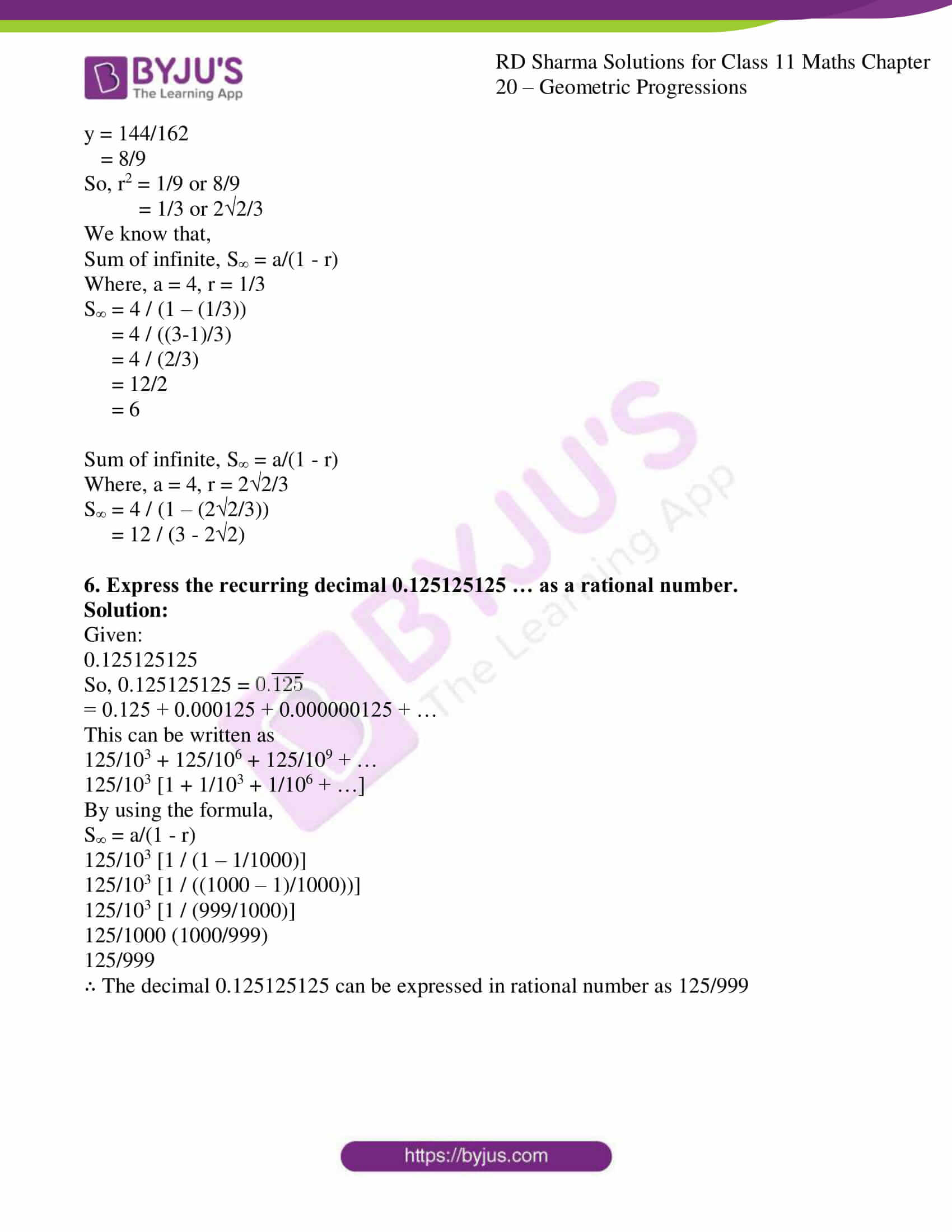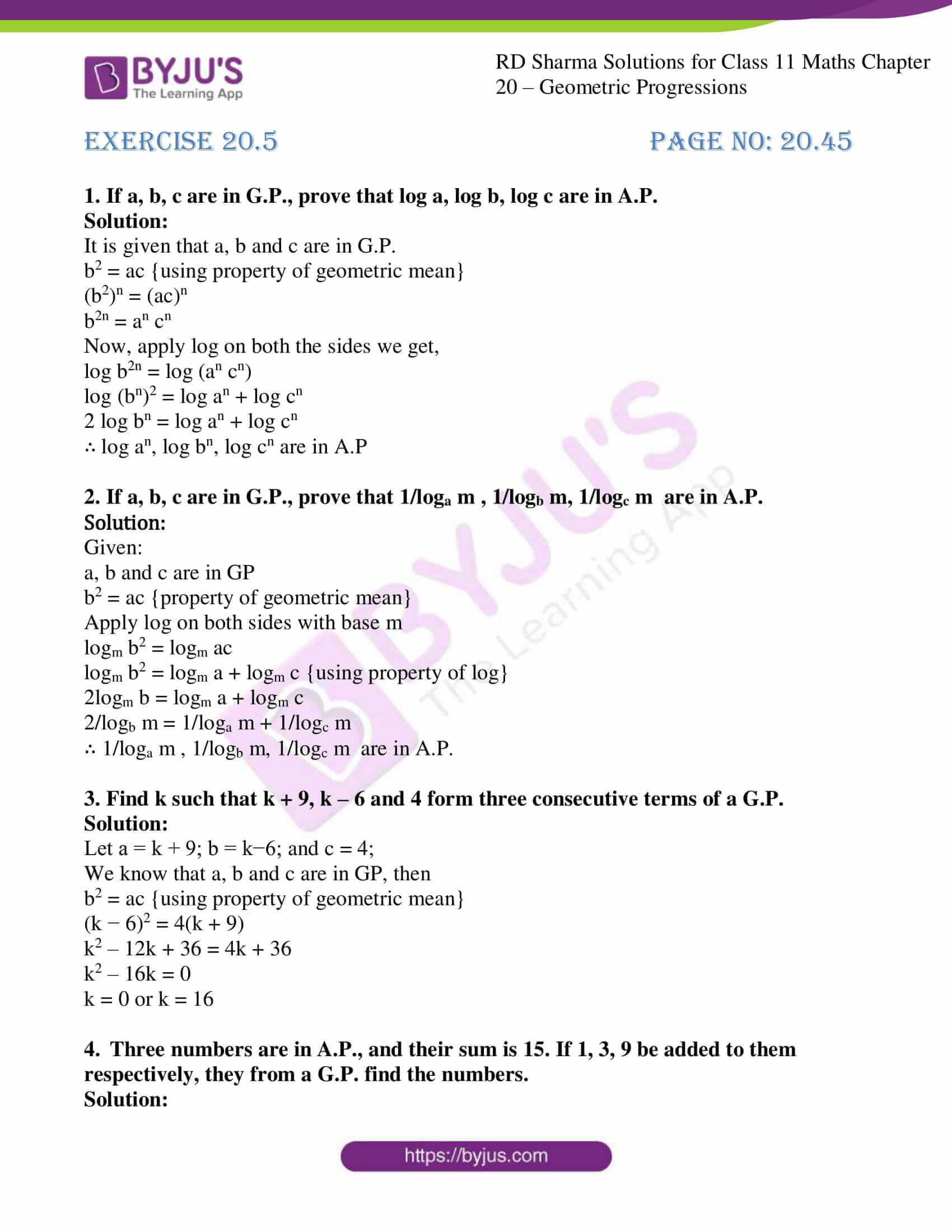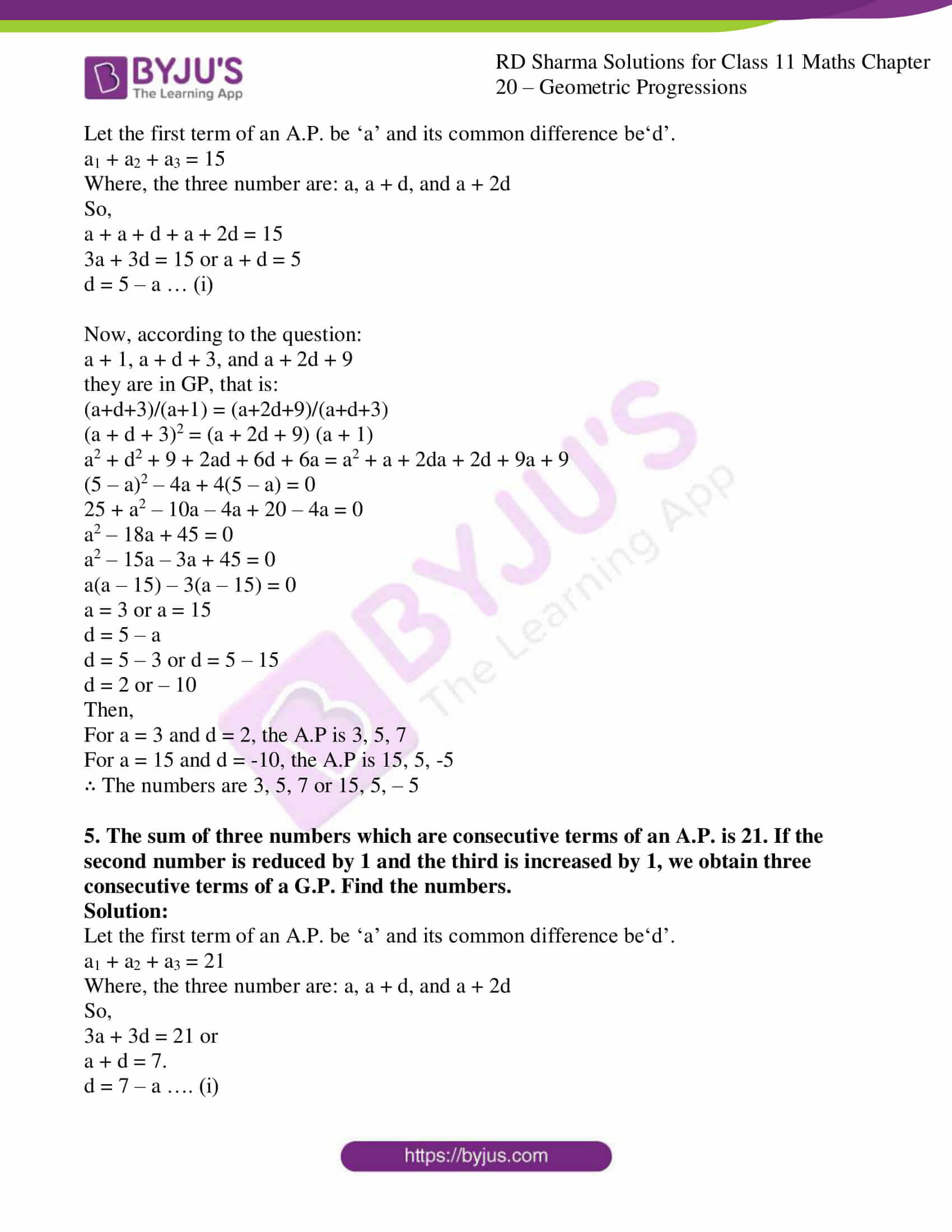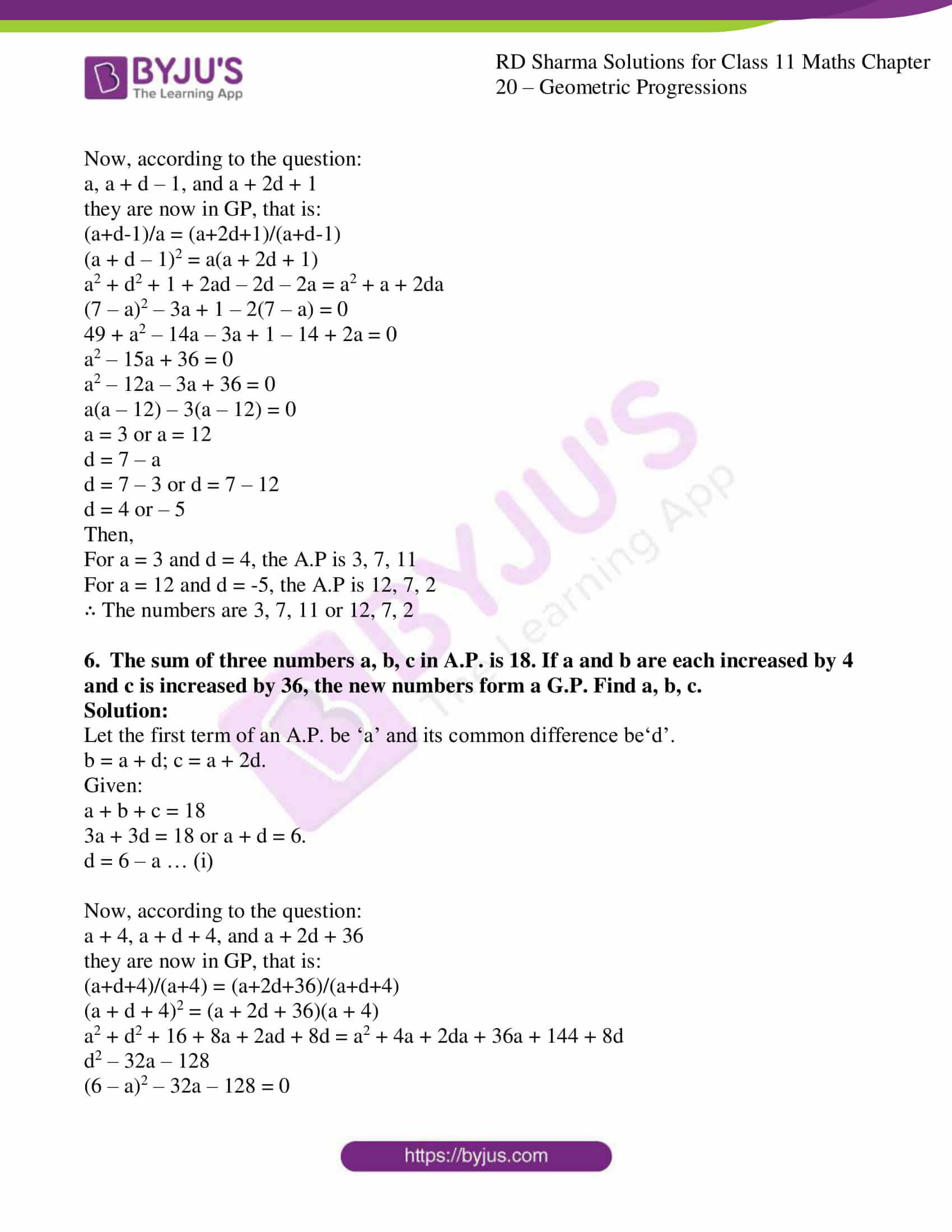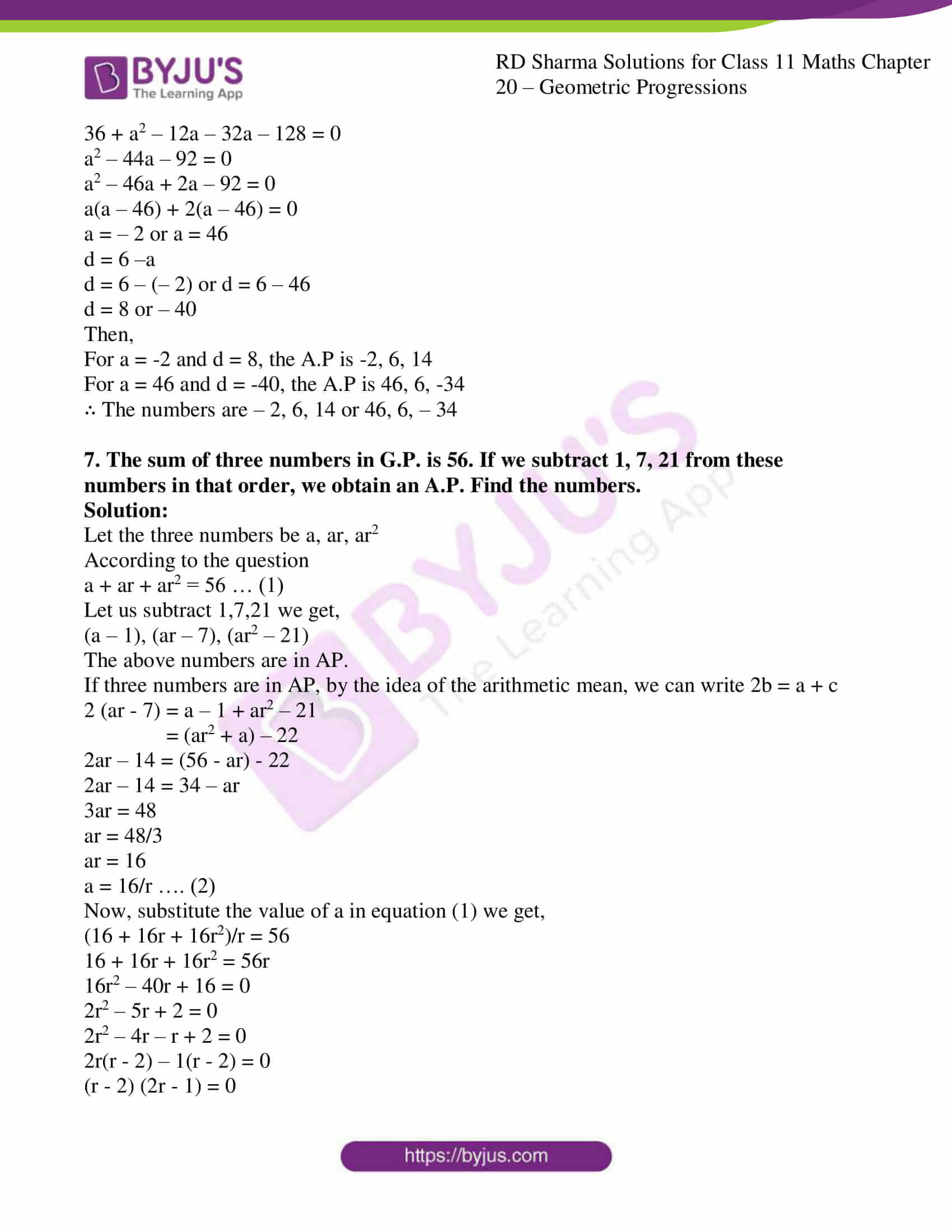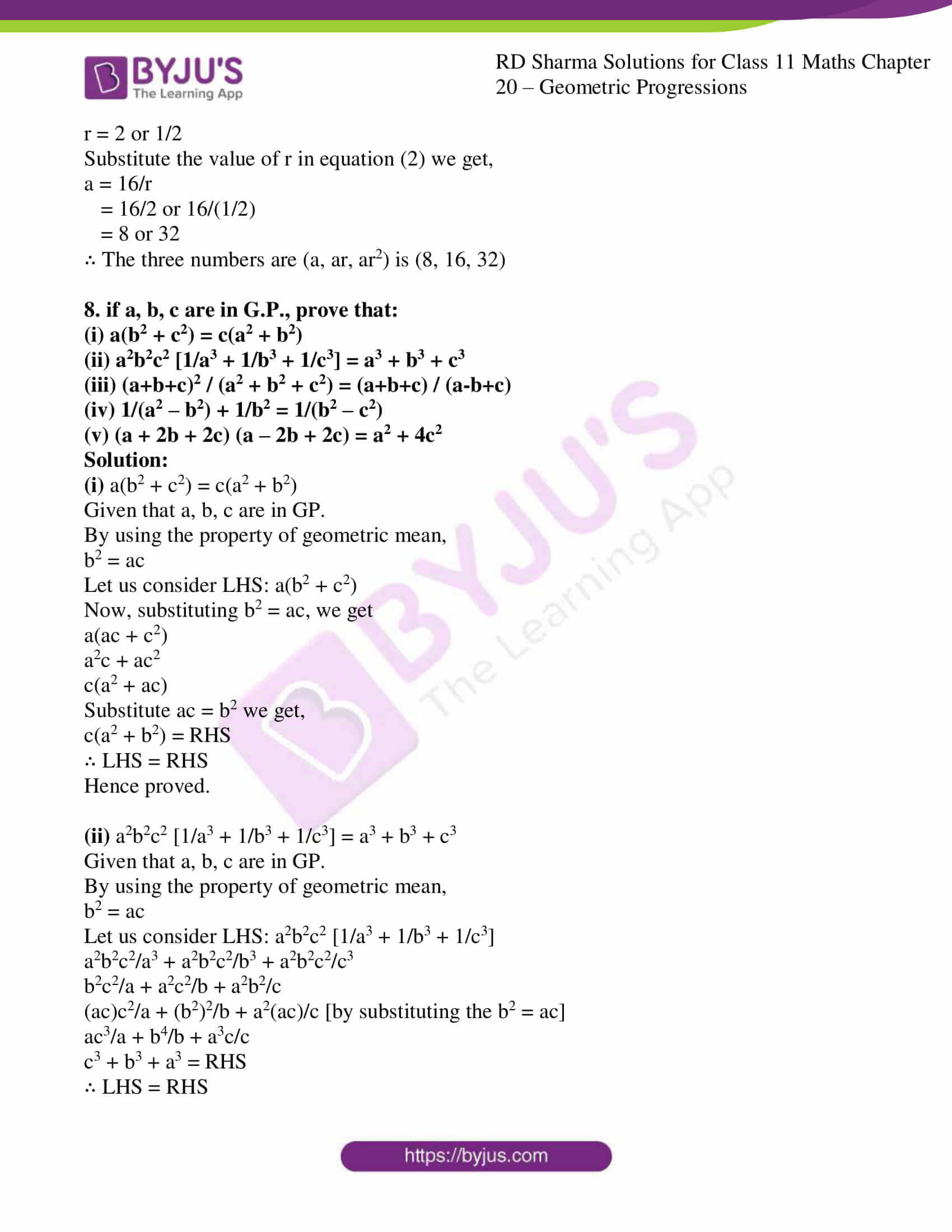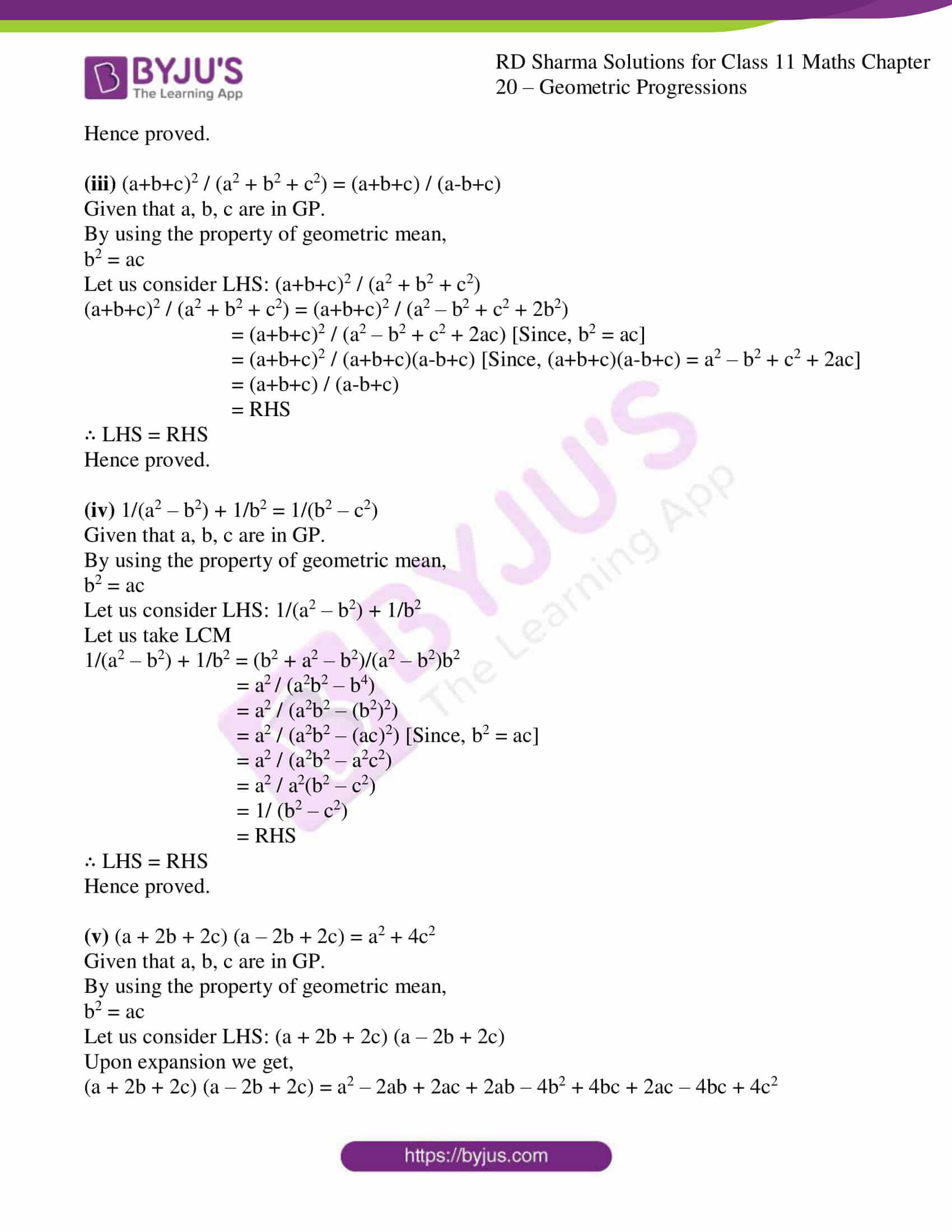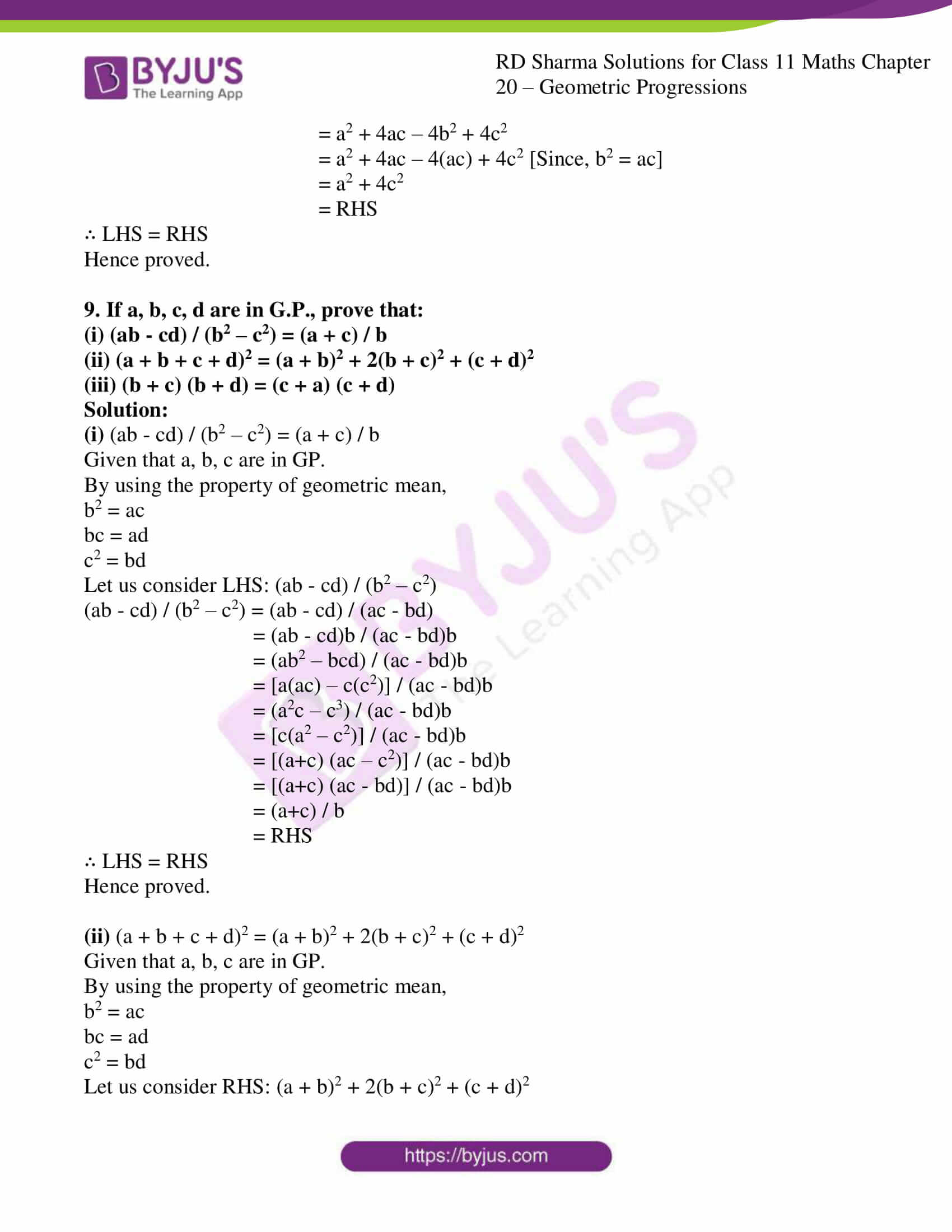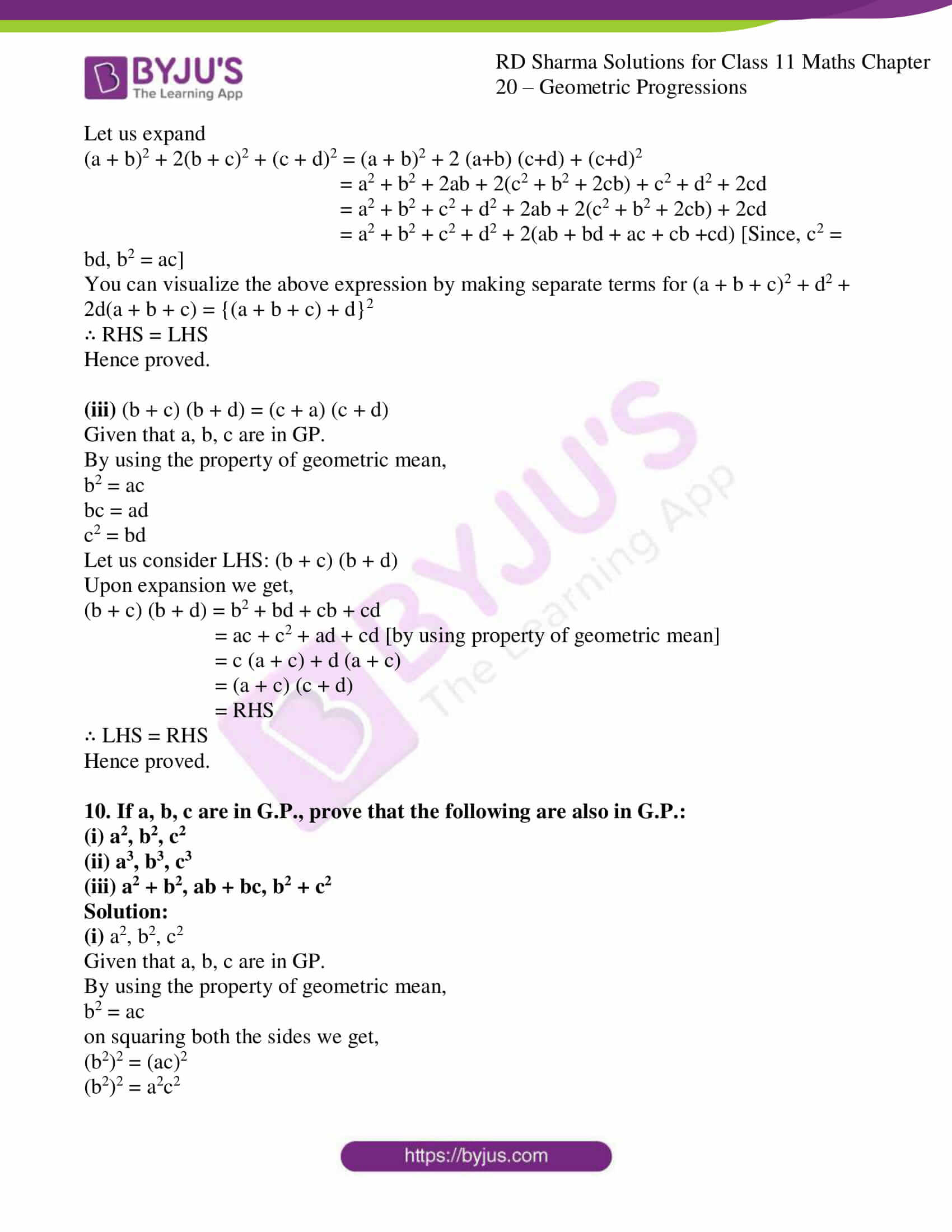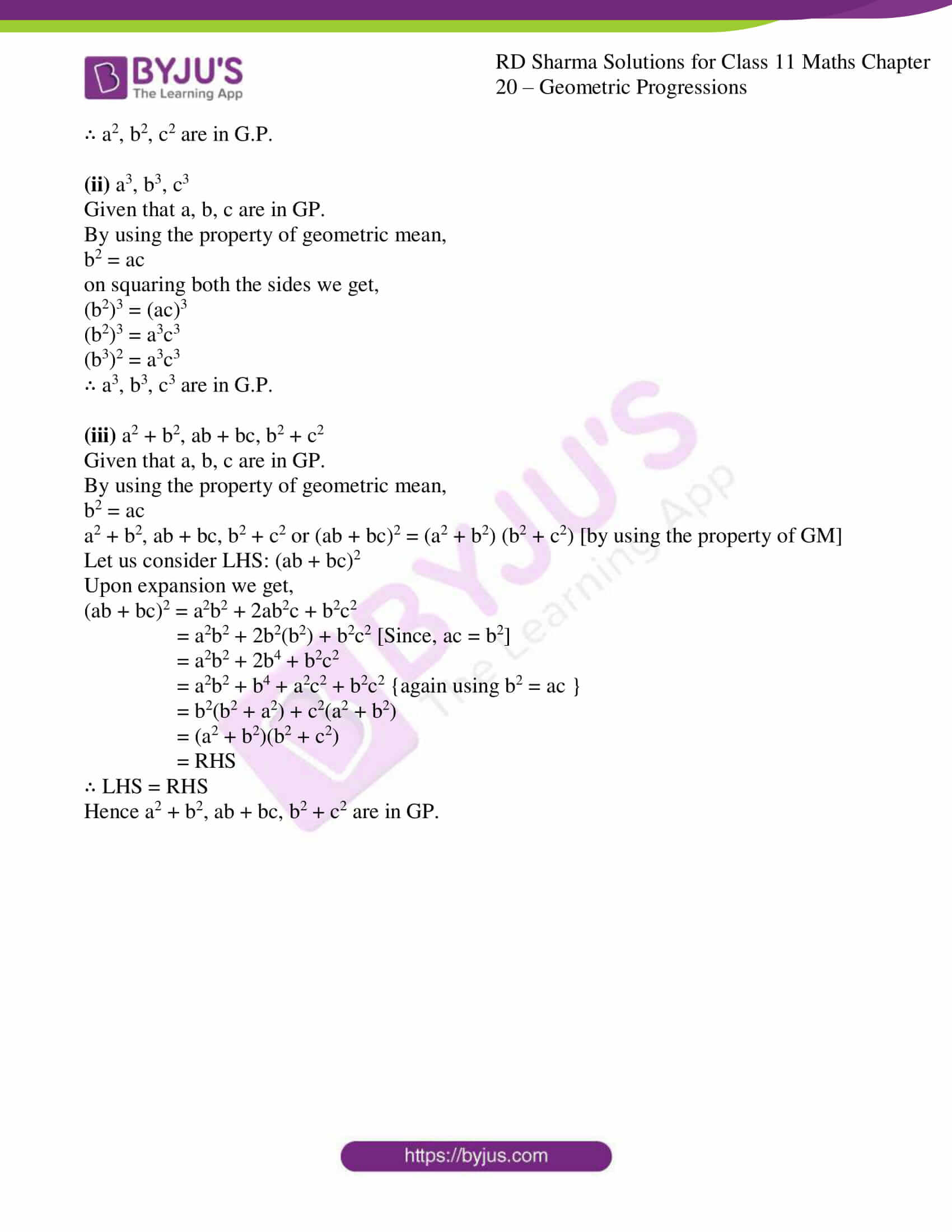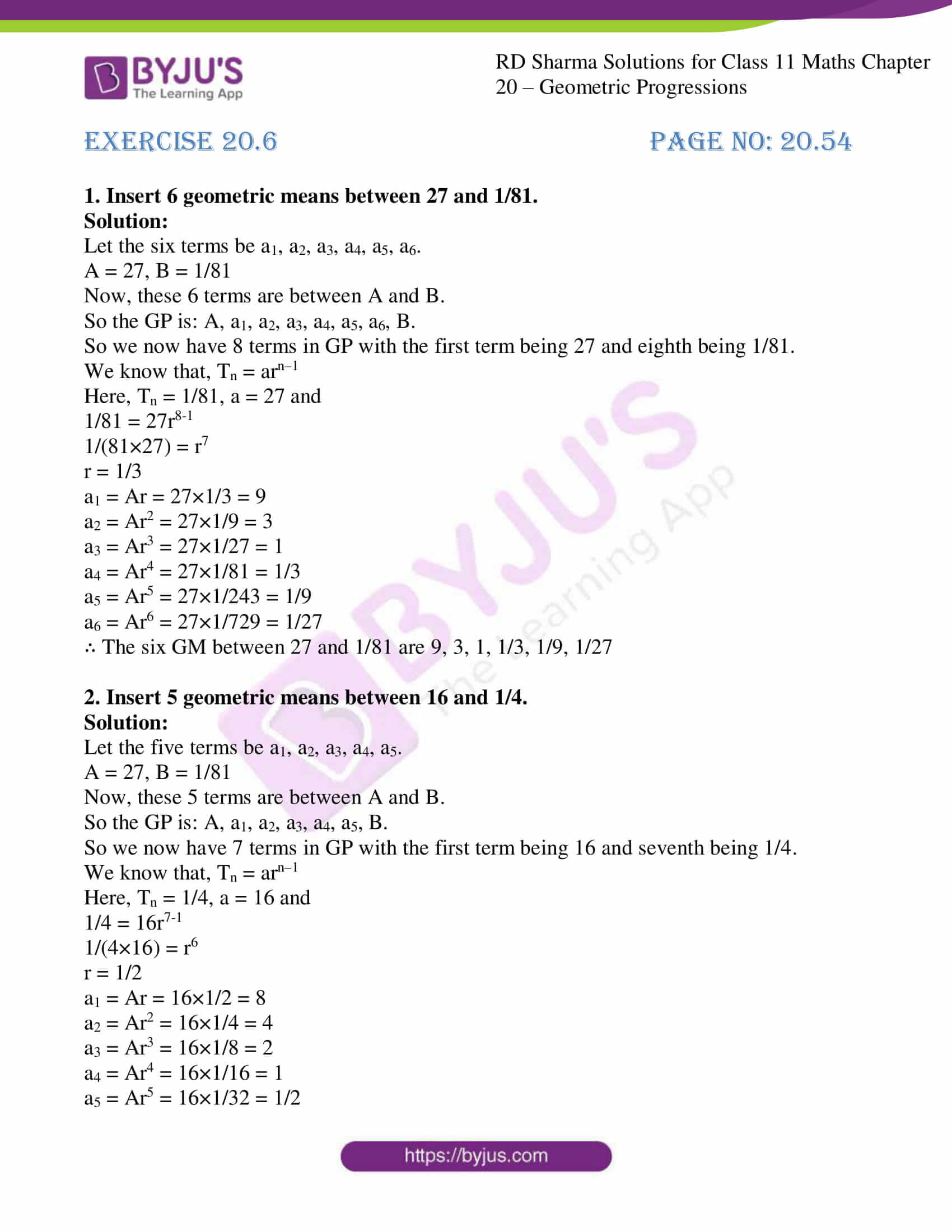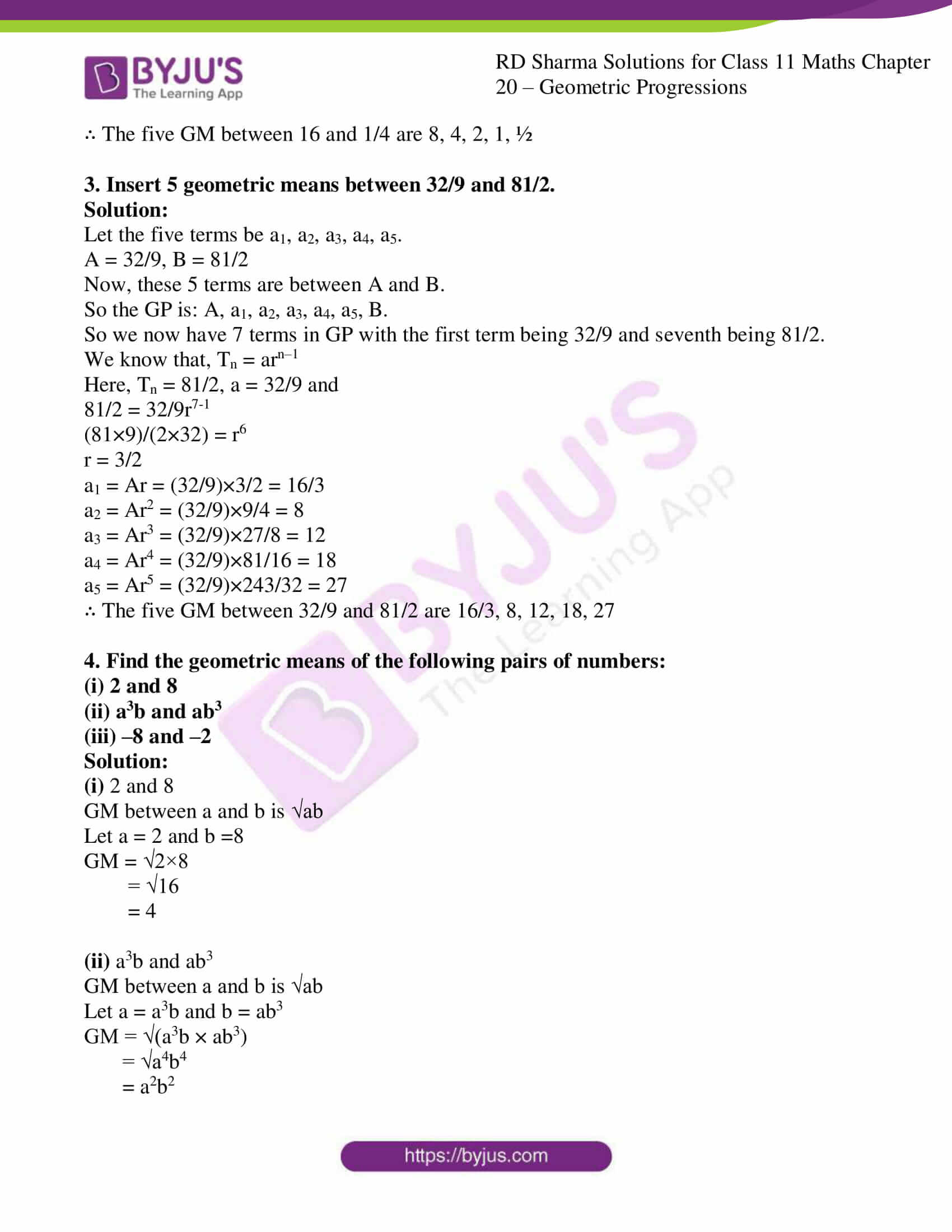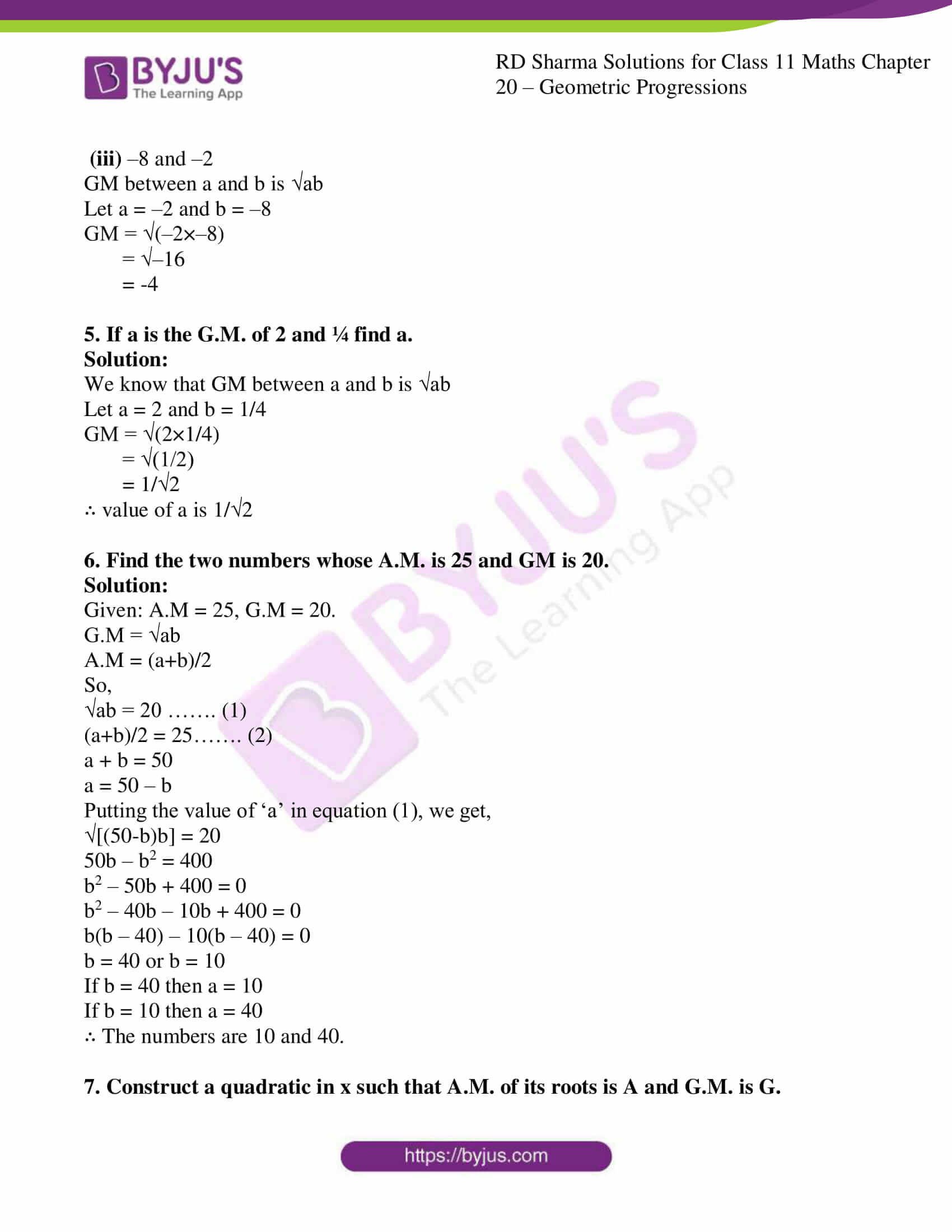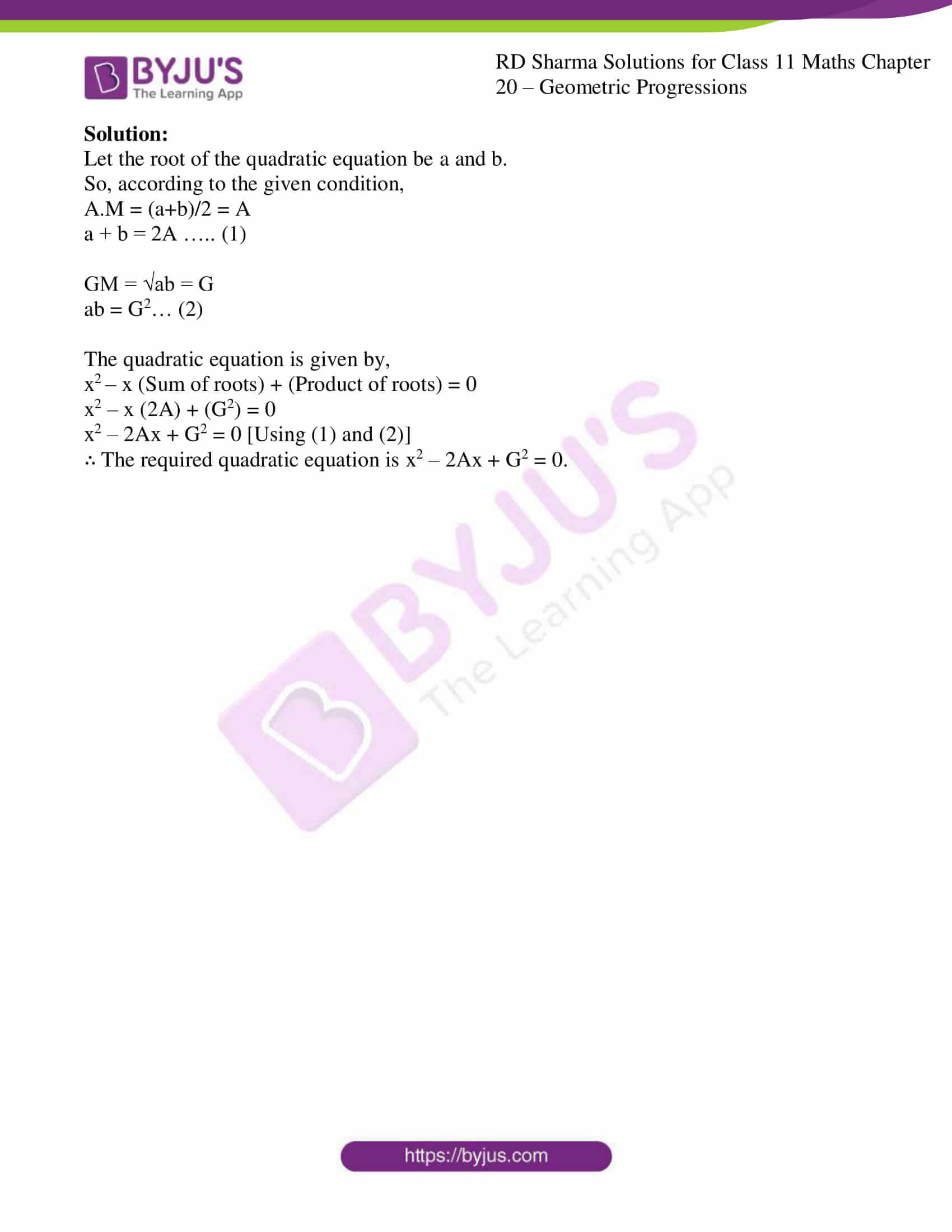### Access answers to RD Sharma Solutions for Class 11 Maths Chapter 20 – Geometric Progressions

EXERCISE 20.1 PAGE NO: 20.9

1. Show that each one of the following progressions is a G.P. Also, find the common ratio in each case:

(i) 4, -2, 1, -1/2, ….

(ii) -2/3, -6, -54, ….

(iii) a, 3a2/4, 9a3/16, ….

(iv) ½, 1/3, 2/9, 4/27, …

Solution:

(i) 4, -2, 1, -1/2, ….

Let a = 4, b = -2, c = 1

In GP,

b2 = ac

(-2)2 = 4(1)

4 = 4

So, the Common ratio = r = -2/4 = -1/2

(ii) -2/3, -6, -54, ….

Let a = -2/3, b = -6, c = -54

In GP,

b2 = ac

(-6)2 = -2/3 × (-54)

36 = 36

So, the Common ratio = r = -6/(-2/3) = -6 × 3/-2 = 9

(iii) a, 3a2/4, 9a3/16, ….

Let a = a, b = 3a2/4, c = 9a3/16

In GP,

b2 = ac

(3a2/4)2 = 9a3/16 × a

9a4/4 = 9a4/16

So, the Common ratio = r = (3a2/4)/a = 3a2/4a = 3a/4

(iv) ½, 1/3, 2/9, 4/27, …

Let a = 1/2, b = 1/3, c = 2/9

In GP,

b2 = ac

(1/3)2 = 1/2 × (2/9)

1/9 = 1/9

So, the Common ratio = r = (1/3)/(1/2) = (1/3) × 2 = 2/3

2. Show that the sequence defined by an = 2/3n, n ∈ N is a G.P.

Solution:

Given:

an = 2/3n

Let us consider n = 1, 2, 3, 4, … since n is a natural number.

So,

a1 = 2/3

a2 = 2/32 = 2/9

a3 = 2/33 = 2/27

a4 = 2/34 = 2/81

In GP,

a3/a2 = (2/27) / (2/9)

= 2/27 × 9/2

= 1/3

a2/a1 = (2/9) / (2/3)

= 2/9 × 3/2

= 1/3

∴ Common ratio of consecutive term is 1/3. Hence n ∈ N is a G.P.

3. Find:

(i) the ninth term of the G.P. 1, 4, 16, 64, ….

(ii) the 10th term of the G.P. -3/4, ½, -1/3, 2/9, ….

(iii) the 8th term of the G.P. 0.3, 0.06, 0.012, ….

(iv) the 12th term of the G.P. 1/a3x3 , ax, a5x5, ….

(v) nth term of the G.P. √3, 1/√3, 1/3√3, …

(vi) the 10th term of the G.P. √2, 1/√2, 1/2√2, ….

Solution:

(i) the ninth term of the G.P. 1, 4, 16, 64, ….

We know that,

t1 = a = 1, r = t2/t1 = 4/1 = 4

By using the formula,

Tn = arn-1

T9 = 1 (4)9-1

= 1 (4)8

= 48

(ii) the 10th term of the G.P. -3/4, ½, -1/3, 2/9, ….

We know that,

t1 = a = -3/4, r = t2/t1 = (1/2) / (-3/4) = ½ × -4/3 = -2/3

By using the formula,

Tn = arn-1

T10 = -3/4 (-2/3)10-1

= -3/4 (-2/3)9

= ½ (2/3)8

(iii) the 8th term of the G.P., 0.3, 0.06, 0.012, ….

We know that,

t1 = a = 0.3, r = t2/t1 = 0.06/0.3 = 0.2

By using the formula,

Tn = arn-1

T8 = 0.3 (0.2)8-1

= 0.3 (0.2)7

(iv) the 12th term of the G.P. 1/a3x3 , ax, a5x5, ….

We know that,

t1 = a = 1/a3x3, r = t2/t1 = ax/(1/a3x3) = ax (a3x3) = a4x4

By using the formula,

Tn = arn-1

T12 = 1/a3x3 (a4x4)12-1

= 1/a3x3 (a4x4)11

= (ax)41

(v) nth term of the G.P. √3, 1/√3, 1/3√3, …

We know that,

t1 = a = √3, r = t2/t1 = (1/√3)/√3 = 1/(√3×√3) = 1/3

By using the formula,

Tn = arn-1

Tn = √3 (1/3)n-1

(vi) the 10th term of the G.P. √2, 1/√2, 1/2√2, ….

We know that,

t1 = a = √2, r = t2/t1 = (1/√2)/√2 = 1/(√2×√2) = 1/2

By using the formula,

Tn = arn-1

T10 = √2 (1/2)10-1

= √2 (1/2)9

= 1/√2 (1/2)8

4. Find the 4th term from the end of the G.P. 2/27, 2/9, 2/3, …., 162.

Solution:

The nth term from the end is given by:

an = l (1/r)n-1 where, l is the last term, r is the common ratio, n is the nth term

Given: last term, l = 162

r = t2/t1 = (2/9) / (2/27)

= 2/9 × 27/2

= 3

n = 4

So, an = l (1/r)n-1

a4 = 162 (1/3)4-1

= 162 (1/3)3

= 162 × 1/27

= 6

∴ 4th term from last is 6.

5. Which term of the progression 0.004, 0.02, 0.1, …. is 12.5?

Solution:

By using the formula,

Tn = arn-1

Given:

a = 0.004

r = t2/t1 = (0.02/0.004)

= 5

Tn = 12.5

n = ?

So, Tn = arn-1

12.5 = (0.004) (5)n-1

12.5/0.004 = 5n-1

3000 = 5n-1

55 = 5n-1

5 = n-1

n = 5 + 1

= 6

∴ 6th term of the progression 0.004, 0.02, 0.1, …. is 12.5.

6. Which term of the G.P.:

(i) √2, 1/√2, 1/2√2, 1/4√2, … is 1/512√2 ?

(ii) 2, 2√2, 4, … is 128 ?

(iii) √3, 3, 3√3, … is 729 ?

(iv) 1/3, 1/9, 1/27… is 1/19683 ?

Solution:

(i) √2, 1/√2, 1/2√2, 1/4√2, … is 1/512√2 ?

By using the formula,

Tn = arn-1

a = √2

r = t2/t1 = (1/√2) / (√2)

= 1/2

Tn = 1/512√2

n = ?

Tn = arn-1

1/512√2 = (√2) (1/2)n-1

1/512√2×√2 = (1/2)n-1

1/512×2 = (1/2)n-1

1/1024 = (1/2)n-1

(1/2)10 = (1/2)n-1

10 = n – 1

n = 10 + 1

= 11

∴ 11th term of the G.P is 1/512√2

(ii) 2, 2√2, 4, … is 128 ?

By using the formula,

Tn = arn-1

a = 2

r = t2/t1 = (2√2/2)

= √2

Tn = 128

n = ?

Tn = arn-1

128 = 2 (√2)n-1

128/2 = (√2)n-1

64 = (√2)n-1

26 = (√2)n-1

12 = n – 1

n = 12 + 1

= 13

∴ 13th term of the G.P is 128

(iii) √3, 3, 3√3, … is 729 ?

By using the formula,

Tn = arn-1

a = √3

r = t2/t1 = (3/√3)

= √3

Tn = 729

n = ?

Tn = arn-1

729 = √3 (√3)n-1

729 = (√3)n

36 = (√3)n

(√3)12 = (√3)n

n = 12

∴ 12th term of the G.P is 729

(iv) 1/3, 1/9, 1/27… is 1/19683 ?

By using the formula,

Tn = arn-1

a = 1/3

r = t2/t1 = (1/9) / (1/3)

= 1/9 × 3/1

= 1/3

Tn = 1/19683

n = ?

Tn = arn-1

1/19683 = (1/3) (1/3)n-1

1/19683 = (1/3)n

(1/3)9 = (1/3)n

n = 9

∴ 9th term of the G.P is 1/19683

7. Which term of the progression 18, -12, 8, … is 512/729 ?

Solution:

By using the formula,

Tn = arn-1

a = 18

r = t2/t1 = (-12/18)

= -2/3

Tn = 512/729

n = ?

Tn = arn-1

512/729 = 18 (-2/3)n-1

29/(729 × 18) = (-2/3)n-1

29/36 × 1/2×32 = (-2/3)n-1

(2/3)8 = (-1)n-1 (2/3)n-1

8 = n – 1

n = 8 + 1

= 9

∴ 9th term of the Progression is 512/729

8. Find the 4th term from the end of the G.P. ½, 1/6, 1/18, 1/54, … , 1/4374

Solution:

The nth term from the end is given by:

an = l (1/r)n-1 where, l is the last term, r is the common ratio, n is the nth term

Given: last term, l = 1/4374

r = t2/t1 = (1/6) / (1/2)

= 1/6 × 2/1

= 1/3

n = 4

So, an = l (1/r)n-1

a4 = 1/4374 (1/(1/3))4-1

= 1/4374 (3/1)3

= 1/4374 × 33

= 1/4374 × 27

= 1/162

∴ 4th term from last is 1/162.

EXERCISE 20.2 PAGE NO: 20.16

1. Find three numbers in G.P. whose sum is 65 and whose product is 3375.

Solution:

Let the three numbers be a/r, a, ar

So, according to the question

a/r + a + ar = 65 … equation (1)

a/r × a × ar = 3375 … equation (2)

From equation (2) we get,

a3 = 3375

a = 15.

From equation (1) we get,

(a + ar + ar2)/r = 65

a + ar + ar2 = 65r … equation (3)

Substituting a = 15 in equation (3) we get

15 + 15r + 15r2 = 65r

15r2 – 50r + 15 = 0… equation (4)

Dividing equation (4) by 5 we get

3r2 – 10r + 3 = 0

3r2 – 9r – r + 3 = 0

3r(r – 3) – 1(r – 3) = 0

r = 3 or r = 1/3

Now, the equation will be

15/3, 15, 15×3 or

15/(1/3), 15, 15×1/3

So the terms are 5, 15, 45 or 45, 15, 5

∴ The three numbers are 5, 15, 45.

2. Find three number in G.P. whose sum is 38 and their product is 1728.

Solution:

Let the three numbers be a/r, a, ar

So, according to the question

a/r + a + ar = 38 … equation (1)

a/r × a × ar = 1728 … equation (2)

From equation (2) we get,

a3 = 1728

a = 12.

From equation (1) we get,

(a + ar + ar2)/r = 38

a + ar + ar2 = 38r … equation (3)

Substituting a = 12 in equation (3) we get

12 + 12r + 12r2 = 38r

12r2 – 26r + 12 = 0… equation (4)

Dividing equation (4) by 2 we get

6r2 – 13r + 6 = 0

6r2 – 9r – 4r + 6 = 0

3r(3r – 3) – 2(3r – 3) = 0

r = 3/2 or r = 2/3

Now the equation will be

12/(3/2) = 8 or

12/(2/3) = 18

So the terms are 8, 12, 18

∴ The three numbers are 8, 12, 18

3. The sum of first three terms of a G.P. is 13/12, and their product is – 1. Find the G.P.

Solution:

Let the three numbers be a/r, a, ar

So, according to the question

a/r + a + ar = 13/12 … equation (1)

a/r × a × ar = -1 … equation (2)

From equation (2) we get,

a3 = -1

a = -1

From equation (1) we get,

(a + ar + ar2)/r = 13/12

12a + 12ar + 12ar2 = 13r … equation (3)

Substituting a = – 1 in equation (3) we get

12( – 1) + 12( – 1)r + 12( – 1)r2 = 13r

12r2 + 25r + 12 = 0

12r2 + 16r + 9r + 12 = 0… equation (4)

4r (3r + 4) + 3(3r + 4) = 0

r = -3/4 or r = -4/3

Now the equation will be

-1/(-3/4), -1, -1×-3/4 or -1/(-4/3), -1, -1×-4/3

4/3, -1, ¾ or ¾, -1, 4/3

∴ The three numbers are 4/3, -1, ¾ or ¾, -1, 4/3

4. The product of three numbers in G.P. is 125 and the sum of their products taken in pairs is 87 ½ . Find them.

Solution:

Let the three numbers be a/r, a, ar

So, according to the question

a/r × a × ar = 125 … equation (1)

From equation (1) we get,

a3 = 125

a = 5

a/r × a + a × ar + ar × a/r = 87 ½

a/r × a + a × ar + ar × a/r = 195/2

a2/r + a2r + a2 = 195/2

a2 (1/r + r + 1) = 195/2

Substituting a = 5 in above equation we get,

52 [(1+r2+r)/r] = 195/2

1+r2+r = (195r/2×25)

2(1+r2+r) = 39r/5

10 + 10r2 + 10r = 39r

10r2 – 29r + 10 = 0

10r2 – 25r – 4r + 10 = 0

5r(2r-5) – 2(2r-5) = 0

r = 5/2, 2/5

So G.P is 10, 5, 5/2 or 5/2, 5, 10

∴ The three numbers are 10, 5, 5/2 or 5/2, 5, 10

5. The sum of the first three terms of a G.P. is 39/10, and their product is 1. Find the common ratio and the terms.

Solution:

Let the three numbers be a/r, a, ar

So, according to the question

a/r + a + ar = 39/10 … equation (1)

a/r × a × ar = 1 … equation (2)

From equation (2) we get,

a3 = 1

a = 1

From equation (1) we get,

(a + ar + ar2)/r = 39/10

10a + 10ar + 10ar2 = 39r … equation (3)

Substituting a = 1 in 3 we get

10(1) + 10(1)r + 10(1)r2 = 39r

10r2 – 29r + 10 = 0

10r2 – 25r – 4r + 10 = 0… equation (4)

5r(2r – 5) – 2(2r – 5) = 0

r = 2/5 or 5/2

so now the equation will be,

1/(2/5), 1, 1×2/5 or 1/(5/2), 1, 1×5/2

5/2, 1, 2/5 or 2/5, 1, 5/2

∴ The three numbers are 2/5, 1, 5/2

EXERCISE 20.3 PAGE NO: 20.27

1. Find the sum of the following geometric progressions:

(i) 2, 6, 18, … to 7 terms

(ii) 1, 3, 9, 27, … to 8 terms

(iii) 1, -1/2, ¼, -1/8, …

(iv) (a2 – b2), (a – b), (a-b)/(a+b), … to n terms

(v) 4, 2, 1, ½ … to 10 terms

Solution:

(i) 2, 6, 18, … to 7 terms

We know that, sum of GP for n terms = a(rn – 1)/(r – 1)

Given:

a = 2, r = t2/t1 = 6/2 = 3, n = 7

Now let us substitute the values in

a(rn – 1)/(r – 1) = 2 (37 – 1)/(3-1)

= 2 (37 – 1)/2

= 37 – 1

= 2187 – 1

= 2186

(ii) 1, 3, 9, 27, … to 8 terms

We know that, sum of GP for n terms = a(rn – 1)/(r – 1)

Given:

a = 1, r = t2/t1 = 3/1 = 3, n = 8

Now let us substitute the values in

a(rn – 1)/(r – 1) = 1 (38 – 1)/(3-1)

= (38 – 1)/2

= (6561 – 1)/2

= 6560/2

= 3280

(iii) 1, -1/2, ¼, -1/8, …

We know that, sum of GP for infinity = a/(1 – r)

Given:

a = 1, r = t2/t1 = (-1/2)/1 = -1/2

Now let us substitute the values in

a/(1 – r) = 1/(1 – (-1/2))

= 1/(1 + 1/2)

= 1/((2+1)/2)

= 1/(3/2)

= 2/3

(iv) (a2 – b2), (a – b), (a-b)/(a+b), … to n terms

We know that, sum of GP for n terms = a(rn – 1)/(r – 1)

Given:

a = (a2 – b2), r = t2/t1 = (a-b)/(a2 – b2) = (a-b)/(a-b) (a+b) = 1/(a+b), n = n

Now let us substitute the values in

a(rn – 1)/(r – 1) =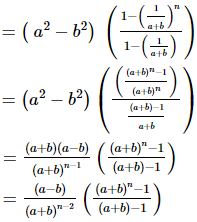(v) 4, 2, 1, ½ … to 10 terms

We know that, sum of GP for n terms = a(rn – 1)/(r – 1)

Given:

a = 4, r = t2/t1 = 2/4 = 1/2, n = 10

Now let us substitute the values in

a(rn – 1)/(r – 1) = 4 ((1/2)10 – 1)/((1/2)-1)

= 4 ((1/2)10 – 1)/((1-2)/2)

= 4 ((1/2)10 – 1)/(-1/2)

= 4 ((1/2)10 – 1) × -2/1

= -8 [1/1024 -1]

= -8 [1 – 1024]/1024

= -8 [-1023]/1024

= 1023/128

2. Find the sum of the following geometric series :
(i) 0.15 + 0.015 + 0.0015 + … to 8 terms;

(ii) √2 + 1/√2 + 1/2√2 + …. to 8 terms;

(iii) 2/9 – 1/3 + ½ – ¾ + … to 5 terms;

(iv) (x + y) + (x2 + xy + y2) + (x3 + x2 y + xy2 + y3) + …. to n terms ;

(v) 3/5 + 4/52 + 3/53 + 4/54 + … to 2n terms;

Solution:

(i) 0.15 + 0.015 + 0.0015 + … to 8 terms

Given:

a = 0.15

r = t2/t1 = 0.015/0.15 = 0.1 = 1/10

n = 8

By using the formula,

Sum of GP for n terms = a(1 – rn )/(1 – r)

a(1 – rn )/(1 – r) = 0.15 (1 – (1/10)8) / (1 – (1/10))

= 0.15 (1 – 1/108) / (1/10)

= 1/6 (1 – 1/108)

(ii) √2 + 1/√2 + 1/2√2 + …. to 8 terms;

Given:

a = √2

r = t2/t1 = (1/√2)/√2 = 1/2

n = 8

By using the formula,

Sum of GP for n terms = a(1 – rn )/(1 – r)

a(1 – rn )/(1 – r) = √2 (1 – (1/2)8) / (1 – (1/2))

= √2 (1 – 1/256) / (1/2)

= √2 ((256 – 1)/256) × 2

= √2 (255×2)/256

= (255√2)/128

(iii) 2/9 – 1/3 + ½ – ¾ + … to 5 terms;

Given:

a = 2/9

r = t2/t1 = (-1/3) / (2/9) = -3/2

n = 5

By using the formula,

Sum of GP for n terms = a(1 – rn )/(1 – r)

a(1 – rn )/(1 – r) = (2/9) (1 – (-3/2)5) / (1 – (-3/2))

= (2/9) (1 + (3/2)5) / (1 + 3/2)

= (2/9) (1 + (3/2)5) / (5/2)

= (2/9) (1 + 243/32) / (5/2)

= (2/9) ((32+243)/32) / (5/2)

= (2/9) (275/32) × 2/5

= 55/72

(iv) (x + y) + (x2 + xy + y2) + (x3 + x2 y + xy2 + y3) + …. to n terms;

Let Sn = (x + y) + (x2 + xy + y2) + (x3 + x2 y + xy2 + y3) + …. to n terms

Let us multiply and divide by (x – y) we get,

Sn = 1/(x – y) [(x + y) (x – y) + (x2 + xy + y2) (x – y) … upto n terms]

(x – y) Sn = (x2 – y2) + x3 + x2y + xy2 – x2y – xy2 – y3..upto n terms

(x – y) Sn = (x2 + x3 + x4+…n terms) – (y2 + y3 + y4 +…n terms)

By using the formula,

Sum of GP for n terms = a(1 – rn )/(1 – r)

We have two G.Ps in above sum, so,

(x – y) Sn = x2 [(xn – 1)/ (x – 1)] – y2 [(yn – 1)/ (y – 1)]

Sn = 1/(x-y) {x2 [(xn – 1)/ (x – 1)] – y2 [(yn – 1)/ (y – 1)]}

(v) 3/5 + 4/52 + 3/53 + 4/54 + … to 2n terms;

The series can be written as:

3 (1/5 + 1/53 + 1/55+ … to n terms) + 4 (1/52 + 1/54 + 1/56 + … to n terms)

Firstly let us consider 3 (1/5 + 1/53 + 1/55+ … to n terms)

So, a = 1/5

r = t2/t1 = 1/52 = 1/25

By using the formula,

Sum of GP for n terms = a(1 – rn )/(1 – r)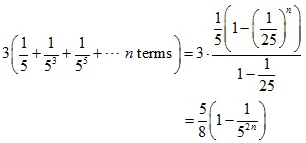Now, Let us consider 4 (1/52 + 1/54 + 1/56 + … to n terms)

So, a = 1/25

r = t2/t1 = 1/52 = 1/25

By using the formula,

Sum of GP for n terms = a(1 – rn )/(1 – r)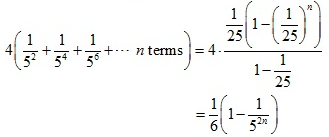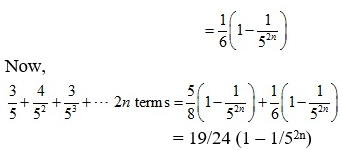3. Evaluate the following: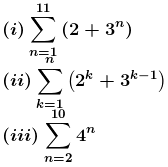Solution: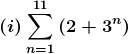= (2 + 31) + (2 + 32) + (2 + 33) + … + (2 + 311)

= 2×11 + 31 + 32 + 33 + … + 311

= 22 + 3(311 – 1)/(3 – 1) [by using the formula, a(1 – rn )/(1 – r)]

= 22 + 3(311 – 1)/2

= [44 + 3(177147 – 1)]/2

= [44 + 3(177146)]/2

= 265741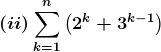= (2 + 30) + (22 + 3) + (23 + 32) + … + (2n + 3n-1)

= (2 + 22 + 23 + … + 2n) + (30 + 31 + 32 + …. + 3n-1)

Firstly let us consider,

(2 + 22 + 23 + … + 2n)

Where, a = 2, r = 22/2 = 4/2 = 2, n = n

By using the formula,

Sum of GP for n terms = a(rn – 1 )/(r – 1)

= 2 (2n – 1)/(2 – 1)

= 2 (2n – 1)

Now, let us consider

(30 + 31 + 32 + …. + 3n)

Where, a = 30 = 1, r = 3/1 = 3, n = n

By using the formula,

Sum of GP for n terms = a(rn – 1 )/(r – 1)

= 1 (3n – 1)/ (3 – 1)

= (3n – 1)/2

So,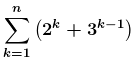= (2 + 22 + 23 + … + 2n) + (30 + 31 + 32 + …. + 3n)

= 2 (2n – 1) + (3n – 1)/2

= ½ [2n+2 + 3n – 4 – 1]

= ½ [2n+2 + 3n – 5]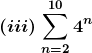= 42 + 43 + 44 + … + 410

Where, a = 42 = 16, r = 43/42 = 4, n = 9

By using the formula,

Sum of GP for n terms = a(rn – 1 )/(r – 1)

= 16 (49 – 1)/(4 – 1)

= 16 (49 – 1)/3

= 16/3 [49 – 1]

4. Find the sum of the following series :
(i) 5 + 55 + 555 + … to n terms.

(ii) 7 + 77 + 777 + … to n terms.

(iii) 9 + 99 + 999 + … to n terms.

(iv) 0.5 + 0.55 + 0.555 + …. to n terms

(v) 0.6 + 0.66 + 0.666 + …. to n terms.

Solution:

(i) 5 + 55 + 555 + … to n terms.

Let us take 5 as a common term so we get,

5 [1 + 11 + 111 + … n terms]

Now multiply and divide by 9 we get,

5/9 [9 + 99 + 999 + … n terms]

5/9 [(10 – 1) + (102 – 1) + (103 – 1) + … n terms]

5/9 [(10 + 102 + 103 + … n terms) – n]

So the G.P is

5/9 [(10 + 102 + 103 + … n terms) – n]

By using the formula,

Sum of GP for n terms = a(rn – 1 )/(r – 1)

Where, a = 10, r = 102/10 = 10, n = n

a(rn – 1 )/(r – 1) =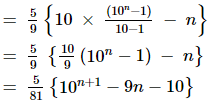(ii) 7 + 77 + 777 + … to n terms.

Let us take 7 as a common term so we get,

7 [1 + 11 + 111 + … to n terms]

Now multiply and divide by 9 we get,

7/9 [9 + 99 + 999 + … n terms]

7/9 [(10 – 1) + (102 – 1) + (103 – 1) + … + (10n – 1)]

7/9 [(10 + 102 + 103 + … +10n)] – 7/9 [(1 + 1 + 1 + … to n terms)]

So the terms are in G.P

Where, a = 10, r = 102/10 = 10, n = n

By using the formula,

Sum of GP for n terms = a(rn – 1 )/(r – 1)

7/9 [10 (10n – 1)/(10-1)] – n

7/9 [10/9 (10n – 1) – n]

7/81 [10 (10n – 1) – n]

7/81 (10n+1 – 9n – 10)

(iii) 9 + 99 + 999 + … to n terms.

The given terms can be written as

(10 – 1) + (100 – 1) + (1000 – 1) + … + n terms

(10 + 102 + 103 + … n terms) – n

By using the formula,

Sum of GP for n terms = a(rn – 1 )/(r – 1)

Where, a = 10, r = 10, n = n

a(rn – 1 )/(r – 1) = [10 (10n – 1)/(10-1)] – n

= 10/9 (10n – 1) – n

= 1/9 [10n+1 – 10 – 9n]

= 1/9 [10n+1 – 9n – 10]

(iv) 0.5 + 0.55 + 0.555 + …. to n terms

Let us take 5 as a common term so we get,

5(0.1 + 0.11 + 0.111 + …n terms)

Now multiply and divide by 9 we get,

5/9 [0.9 + 0.99 + 0.999 + …+ to n terms]

5/9 [9/10 + 9/100 + 9/1000 + … + n terms]

This can be written as

5/9 [(1 – 1/10) + (1 – 1/100) + (1 – 1/1000) + … + n terms]

5/9 [n – {1/10 + 1/102 + 1/103 + … + n terms}]

5/9 [n – 1/10 {1-(1/10)n}/{1 – 1/10}]

5/9 [n – 1/9 (1 – 1/10n)]

(v) 0.6 + 0.66 + 0.666 + …. to n terms.

Let us take 6 as a common term so we get,

6(0.1 + 0.11 + 0.111 + …n terms)

Now multiply and divide by 9 we get,

6/9 [0.9 + 0.99 + 0.999 + …+ n terms]

6/9 [9/10 + 9/100 + 9/1000 + …+ n terms]

This can be written as

6/9 [(1 – 1/10) + (1 – 1/100) + (1 – 1/1000) + … + n terms]

6/9 [n – {1/10 + 1/102 + 1/103 + … + n terms}]

6/9 [n – 1/10 {1-(1/10)n}/{1 – 1/10}]

6/9 [n – 1/9 (1 – 1/10n)]

5. How many terms of the G.P. 3, 3/2, ¾, … Be taken together to make 3069/512 ?

Solution:

Given:

Sum of G.P = 3069/512

Where, a = 3, r = (3/2)/3 = 1/2, n = ?

By using the formula,

Sum of GP for n terms = a(rn – 1 )/(r – 1)

3069/512 = 3 ((1/2)n – 1)/ (1/2 – 1)

3069/512×3×2 = 1 – (1/2)n

3069/3072 – 1 = – (1/2)n

(3069 – 3072)/3072 = – (1/2)n

-3/3072 = – (1/2)n

1/1024 = (1/2)n

(1/2)10 = (1/2)n

10 = n

∴ 10 terms are required to make 3069/512

6. How many terms of the series 2 + 6 + 18 + …. Must be taken to make the sum equal to 728?

Solution:

Given:

Sum of GP = 728

Where, a = 2, r = 6/2 = 3, n = ?

By using the formula,

Sum of GP for n terms = a(rn – 1 )/(r – 1)

728 = 2 (3n – 1)/(3-1)

728 = 2 (3n – 1)/2

728 = 3n – 1

729 = 3n

36 = 3n

6 = n

∴ 6 terms are required to make a sum equal to 728

7. How many terms of the sequence √3, 3, 3√3,… must be taken to make the sum 39+ 13√3 ?

Solution:

Given:

Sum of GP = 39 + 13√3

Where, a =√3, r = 3/√3 = √3, n = ?

By using the formula,

Sum of GP for n terms = a(rn – 1 )/(r – 1)

39 + 13√3 = √3 (√3n – 1)/ (√3 – 1)

(39 + 13√3) (√3 – 1) = √3 (√3n – 1)

Let us simplify we get,

39√3 – 39 + 13(3) – 13√3 = √3 (√3n – 1)

39√3 – 39 + 39 – 13√3 = √3 (√3n – 1)

39√3 – 39 + 39 – 13√3 = √3n+1 – √3

26√3 + √3 = √3n+1

27√3 = √3n+1

√36 √3 = √3n+1

6+1 = n + 1

7 = n + 1

7 – 1 = n

6 = n

∴ 6 terms are required to make a sum of 39 + 13√3

8. The sum of n terms of the G.P. 3, 6, 12, … is 381. Find the value of n.

Solution:

Given:

Sum of GP = 381

Where, a = 3, r = 6/3 = 2, n = ?

By using the formula,

Sum of GP for n terms = a(rn – 1 )/(r – 1)

381 = 3 (2n – 1)/ (2-1)

381 = 3 (2n – 1)

381/3 = 2n – 1

127 = 2n – 1

127 + 1 = 2n

128 = 2n

27 = 2n

n = 7

∴ value of n is 7

9. The common ratio of a G.P. is 3, and the last term is 486. If the sum of these terms be 728, find the first term.

Solution:

Given:

Sum of GP = 728

Where, r = 3, a = ?

Firstly,

Tn = arn-1

486 = a3n-1

486 = a3n/3

486 (3) = a3n

1458 = a3n …. Equation (i)

By using the formula,

Sum of GP for n terms = a(rn – 1 )/(r – 1)

728 = a (3n – 1)/2

1456 = a3n – a … equation (2)

Subtracting equation (1) from (2) we get

1458 – 1456 = a.3n – a.3n + a

a = 2.

∴ The first term is 2

10. The ratio of the sum of the first three terms is to that of the first 6 terms of a G.P. is 125 : 152. Find the common ratio.

Solution:

Given:

Sum of G.P of 3 terms is 125

By using the formula,

Sum of GP for n terms = a(rn – 1 )/(r – 1)

125 = a (rn – 1)/(r-1)

125 = a (r3 – 1)/ (r-1) … equation (1)

Now,

Sum of G.P of 6 terms is 152

By using the formula,

Sum of GP for n terms = a(rn – 1 )/(r – 1)

152 = a (rn – 1)/(r-1)

152 = a (r6 – 1)/ (r-1) … equation (2)

Let us divide equation (i) by (ii) we get,

125/152 = [a (r3 – 1)/ (r-1)] / [a (r6 – 1)/ (r-1)]

125/152 = (r3 – 1)/(r6 – 1)

125/152 = (r3 – 1)/[(r3 – 1) (r3 + 1)]

125/152 = 1/(r3 + 1)

125(r3 + 1) = 152

125r3 + 125 = 152

125r3 = 152 – 125

125r3 = 27

r3 = 27/125

r3 = 33/53

r = 3/5

∴ The common ratio is 3/5

EXERCISE 20.4 PAGE NO: 20.39

1. Find the sum of the following series to infinity:

(i) 1 – 1/3 + 1/32 – 1/33 + 1/34 + … ∞

(ii) 8 + 4√2 + 4 + …. ∞

(iii) 2/5 + 3/52 + 2/53 + 3/54 + …. ∞

(iv) 10 – 9 + 8.1 – 7.29 + …. ∞

Solution:

(i) 1 – 1/3 + 1/32 – 1/33 + 1/34 + … ∞

Given:

S = 1 – 1/3 + 1/32 – 1/33 + 1/34 + … ∞

Where, a = 1, r = -1/3

By using the formula,

S = a/(1 – r)

= 1 / (1 – (-1/3))

= 1/ (1 + 1/3)

= 1/ ((3+1)/3)

= 1/ (4/3)

= ¾

(ii) 8 + 4√2 + 4 + …. ∞

Given:

S = 8 + 4√2 + 4 + …. ∞

Where, a = 8, r = 4/4√2 = 1/√2

By using the formula,

S = a/(1 – r)

= 8 / (1 – (1/√2))

= 8 / ((√2 – 1)/√2)

= 8√2 /(√2 – 1)

Multiply and divide with √2 + 1 we get,

= 8√2 /(√2 – 1) × (√2 + 1)/( √2 + 1)

= 8 (2 + √2)/(2-1)

= 8 (2 + √2)

(iii) 2/5 + 3/52 + 2/53 + 3/54 + …. ∞

The given terms can be written as,

(2/5 + 2/53 + …) + (3/52 + 3/54 + …)

(a = 2/5, r = 1/25) and (a = 3/25, r = 1/25)

By using the formula,

S = a/(1 – r)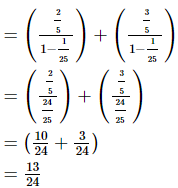(iv) 10 – 9 + 8.1 – 7.29 + …. ∞

Given:

S = 8 + 4√2 + 4 + …. ∞

Where, a = 10, r = -9/10

By using the formula,

S = a/(1 – r)

= 10 / (1 – (-9/10))

= 10 / (1 + 9/10)

= 10 / ((10+9)/10)

= 10 / (19/10)

= 100/19

= 5.263

2. Prove that :
(91/3 . 91/9 . 91/27 ….∞) = 3.

Solution:

Let us consider the LHS

(91/3 . 91/9 . 91/27 ….∞)

This can be written as

91/3 + 1/9 + 1/27 + …∞

So let us consider m = 1/3 + 1/9 + 1/27 + … ∞

Where, a = 1/3, r = (1/9) / (1/3) = 1/3

By using the formula,

S = a/(1 – r)

= (1/3) / (1 – (1/3))

= (1/3) / ((3-1)/3)

= (1/3) / (2/3)

= ½

So, 9m = 91/2 = 3 = RHS

Hence proved.

3. Prove that :

(21/4 .41/8 . 81/16. 161/32….∞) = 2.

Solution:

Let us consider the LHS

(21/4 .41/8 . 81/16. 161/32….∞)

This can be written as

21/4 . 22/8 . 23/16 . 21/8 … ∞

Now,

21/4 + 2/8 + 3/16 + 1/8 + …∞

So let us consider 2x, where x = ¼ + 2/8 + 3/16 + 1/8 + … ∞ …. (1)

Multiply both sides of the equation with 1/2, we get

x/2 = ½ (¼ + 2/8 + 3/16 + 1/8 + … ∞)

= 1/8 + 2/16 + 3/32 + … + ∞ …. (2)

Now, subtract (2) from (1) we get,

x – x/2 = (¼ + 2/8 + 3/16 + 1/8 + … ∞) – (1/8 + 2/16 + 3/32 + … + ∞)

By grouping similar terms,

x/2 = ¼ + (2/8 – 1/8) + (3/16 – 2/16) + … ∞

x/2 = ¼ + 1/8 + 1/16 + … ∞

x = ½ + ¼ + 1/8 + 1/16 + … ∞

Where, a = 1/2, r = (1/4) / (1/2) = 1/2

By using the formula,

S = a/(1 – r)

= (1/2) / (1 – 1/2)

= (1/2) / ((2-1)/2)

= (1/2) / (1/2)

= 1

From equation (1), 2x = 21 = 2 = RHS

Hence proved.

4. If Sp denotes the sum of the series 1 + rp + r2p + … to ∞ and sp the sum of the series 1 – rp + r2p – … to ∞, prove that sp + Sp = 2 S2p.

Solution:

Given:

Sp = 1 + rp + r2p + … ∞

By using the formula,

S = a/(1 – r)

Where, a = 1, r = rp

So,

Sp = 1 / (1 – rp)

Similarly, sp = 1 – rp + r2p – … ∞

By using the formula,

S = a/(1 – r)

Where, a = 1, r = -rp

So,

Sp = 1 / (1 – (-rp))

= 1 / (1 + rp)

Now, Sp + sp = [1 / (1 – rp)] + [1 / (1 + rp)]

2S2p = [(1 – rp) + (1 + rp)] / (1 – r2p)

= 2 /(1 – r2p)

∴ 2S2p = Sp + Sp

5. Find the sum of the terms of an infinite decreasing G.P. in which all the terms are positive, the first term is 4, and the difference between the third and fifth term is equal to 32/81.

Solution:

Let ‘a’ be the first term of GP and ‘r’ be the common ratio.

We know that nth term of a GP is given by-

an = arn-1

As, a = 4 (given)

And a5 – a3 = 32/81 (given)

4r4 – 4r2 = 32/81

4r2(r2 – 1) = 32/81

r2(r2 – 1) = 8/81

Let us denote r2 with y

81y(y-1) = 8

81y2 – 81y – 8 = 0

Using the formula of the quadratic equation to solve the equation, we get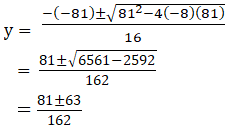y = 18/162 = 1/9 or

y = 144/162

= 8/9

So, r2 = 1/9 or 8/9

= 1/3 or 22/3

We know that,

Sum of infinite, S = a/(1 – r)

Where, a = 4, r = 1/3

S = 4 / (1 – (1/3))

= 4 / ((3-1)/3)

= 4 / (2/3)

= 12/2

= 6

Sum of infinite, S = a/(1 – r)

Where, a = 4, r = 22/3

S = 4 / (1 – (22/3))

= 12 / (3 – 22)

6. Express the recurring decimal 0.125125125 … as a rational number.

Solution:

Given:

0.125125125

So, 0.125125125 =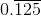= 0.125 + 0.000125 + 0.000000125 + …

This can be written as

125/103 + 125/106 + 125/109 + …

125/103 [1 + 1/103 + 1/106 + …]

By using the formula,

S = a/(1 – r)

125/103 [1 / (1 – 1/1000)]

125/103 [1 / ((1000 – 1)/1000))]

125/103 [1 / (999/1000)]

125/1000 (1000/999)

125/999

∴ The decimal 0.125125125 can be expressed in rational number as 125/999

EXERCISE 20.5 PAGE NO: 20.45

1. If a, b, c are in G.P., prove that log a, log b, log c are in A.P.

Solution:

It is given that a, b and c are in G.P.

b2 = ac {using property of geometric mean}

(b2)n = (ac)n

b2n = an cn

Now, apply log on both the sides we get,

log b2n = log (an cn)

log (bn)2 = log an + log cn

2 log bn = log an + log cn

∴ log an, log bn, log cn are in A.P

2. If a, b, c are in G.P., prove that 1/loga m , 1/logb m, 1/logc m are in A.P.

Solution:

Given:

a, b and c are in GP

b2 = ac {property of geometric mean}

Apply log on both sides with base m

logm b2 = logm ac

logm b2 = logm a + logm c {using property of log}

2logm b = logm a + logm c

2/logb m = 1/loga m + 1/logc m

∴ 1/loga m , 1/logb m, 1/logc m are in A.P.

3. Find k such that k + 9, k – 6 and 4 form three consecutive terms of a G.P.

Solution:

Let a = k + 9; b = k−6; and c = 4;

We know that a, b and c are in GP, then

b2 = ac {using property of geometric mean}

(k − 6)2 = 4(k + 9)

k2 – 12k + 36 = 4k + 36

k2 – 16k = 0

k = 0 or k = 16

4. Three numbers are in A.P., and their sum is 15. If 1, 3, 9 be added to them respectively, they from a G.P. find the numbers.

Solution:

Let the first term of an A.P. be ‘a’ and its common difference be‘d’.

a1 + a2 + a3 = 15

Where, the three number are: a, a + d, and a + 2d

So,

a + a + d + a + 2d = 15

3a + 3d = 15 or a + d = 5

d = 5 – a … (i)

Now, according to the question:

a + 1, a + d + 3, and a + 2d + 9

they are in GP, that is:

(a+d+3)/(a+1) = (a+2d+9)/(a+d+3)

(a + d + 3)2 = (a + 2d + 9) (a + 1)

a2 + d2 + 9 + 2ad + 6d + 6a = a2 + a + 2da + 2d + 9a + 9

(5 – a)2 – 4a + 4(5 – a) = 0

25 + a2 – 10a – 4a + 20 – 4a = 0

a2 – 18a + 45 = 0

a2 – 15a – 3a + 45 = 0

a(a – 15) – 3(a – 15) = 0

a = 3 or a = 15

d = 5 – a

d = 5 – 3 or d = 5 – 15

d = 2 or – 10

Then,

For a = 3 and d = 2, the A.P is 3, 5, 7

For a = 15 and d = -10, the A.P is 15, 5, -5

∴ The numbers are 3, 5, 7 or 15, 5, – 5

5. The sum of three numbers which are consecutive terms of an A.P. is 21. If the second number is reduced by 1 and the third is increased by 1, we obtain three consecutive terms of a G.P. Find the numbers.

Solution:

Let the first term of an A.P. be ‘a’ and its common difference be‘d’.

a1 + a2 + a3 = 21

Where, the three number are: a, a + d, and a + 2d

So,

3a + 3d = 21 or

a + d = 7.

d = 7 – a …. (i)

Now, according to the question:

a, a + d – 1, and a + 2d + 1

they are now in GP, that is:

(a+d-1)/a = (a+2d+1)/(a+d-1)

(a + d – 1)2 = a(a + 2d + 1)

a2 + d2 + 1 + 2ad – 2d – 2a = a2 + a + 2da

(7 – a)2 – 3a + 1 – 2(7 – a) = 0

49 + a2 – 14a – 3a + 1 – 14 + 2a = 0

a2 – 15a + 36 = 0

a2 – 12a – 3a + 36 = 0

a(a – 12) – 3(a – 12) = 0

a = 3 or a = 12

d = 7 – a

d = 7 – 3 or d = 7 – 12

d = 4 or – 5

Then,

For a = 3 and d = 4, the A.P is 3, 7, 11

For a = 12 and d = -5, the A.P is 12, 7, 2

∴ The numbers are 3, 7, 11 or 12, 7, 2

6. The sum of three numbers a, b, c in A.P. is 18. If a and b are each increased by 4 and c is increased by 36, the new numbers form a G.P. Find a, b, c.

Solution:

Let the first term of an A.P. be ‘a’ and its common difference be‘d’.

b = a + d; c = a + 2d.

Given:

a + b + c = 18

3a + 3d = 18 or a + d = 6.

d = 6 – a … (i)

Now, according to the question:

a + 4, a + d + 4, and a + 2d + 36

they are now in GP, that is:

(a+d+4)/(a+4) = (a+2d+36)/(a+d+4)

(a + d + 4)2 = (a + 2d + 36)(a + 4)

a2 + d2 + 16 + 8a + 2ad + 8d = a2 + 4a + 2da + 36a + 144 + 8d

d2 – 32a – 128

(6 – a)2 – 32a – 128 = 0

36 + a2 – 12a – 32a – 128 = 0

a2 – 44a – 92 = 0

a2 – 46a + 2a – 92 = 0

a(a – 46) + 2(a – 46) = 0

a = – 2 or a = 46

d = 6 –a

d = 6 – (– 2) or d = 6 – 46

d = 8 or – 40

Then,

For a = -2 and d = 8, the A.P is -2, 6, 14

For a = 46 and d = -40, the A.P is 46, 6, -34

∴ The numbers are – 2, 6, 14 or 46, 6, – 34

7. The sum of three numbers in G.P. is 56. If we subtract 1, 7, 21 from these numbers in that order, we obtain an A.P. Find the numbers.

Solution:

Let the three numbers be a, ar, ar2

According to the question

a + ar + ar2 = 56 … (1)

Let us subtract 1,7,21 we get,

(a – 1), (ar – 7), (ar2 – 21)

The above numbers are in AP.

If three numbers are in AP, by the idea of the arithmetic mean, we can write 2b = a + c

2 (ar – 7) = a – 1 + ar2 – 21

= (ar2 + a) – 22

2ar – 14 = (56 – ar) – 22

2ar – 14 = 34 – ar

3ar = 48

ar = 48/3

ar = 16

a = 16/r …. (2)

Now, substitute the value of a in equation (1) we get,

(16 + 16r + 16r2)/r = 56

16 + 16r + 16r2 = 56r

16r2 – 40r + 16 = 0

2r2 – 5r + 2 = 0

2r2 – 4r – r + 2 = 0

2r(r – 2) – 1(r – 2) = 0

(r – 2) (2r – 1) = 0

r = 2 or 1/2

Substitute the value of r in equation (2) we get,

a = 16/r

= 16/2 or 16/(1/2)

= 8 or 32

∴ The three numbers are (a, ar, ar2) is (8, 16, 32)

8. if a, b, c are in G.P., prove that:

(i) a(b2 + c2) = c(a2 + b2)

(ii) a2b2c2 [1/a3 + 1/b3 + 1/c3] = a3 + b3 + c3

(iii) (a+b+c)2 / (a2 + b2 + c2) = (a+b+c) / (a-b+c)

(iv) 1/(a2 – b2) + 1/b2 = 1/(b2 – c2)

(v) (a + 2b + 2c) (a – 2b + 2c) = a2 + 4c2

Solution:

(i) a(b2 + c2) = c(a2 + b2)

Given that a, b, c are in GP.

By using the property of geometric mean,

b2 = ac

Let us consider LHS: a(b2 + c2)

Now, substituting b2 = ac, we get

a(ac + c2)

a2c + ac2

c(a2 + ac)

Substitute ac = b2 we get,

c(a2 + b2) = RHS

∴ LHS = RHS

Hence proved.

(ii) a2b2c2 [1/a3 + 1/b3 + 1/c3] = a3 + b3 + c3

Given that a, b, c are in GP.

By using the property of geometric mean,

b2 = ac

Let us consider LHS: a2b2c2 [1/a3 + 1/b3 + 1/c3]

a2b2c2/a3 + a2b2c2/b3 + a2b2c2/c3

b2c2/a + a2c2/b + a2b2/c

(ac)c2/a + (b2)2/b + a2(ac)/c [by substituting the b2 = ac]

ac3/a + b4/b + a3c/c

c3 + b3 + a3 = RHS

∴ LHS = RHS

Hence proved.

(iii) (a+b+c)2 / (a2 + b2 + c2) = (a+b+c) / (a-b+c)

Given that a, b, c are in GP.

By using the property of geometric mean,

b2 = ac

Let us consider LHS: (a+b+c)2 / (a2 + b2 + c2)

(a+b+c)2 / (a2 + b2 + c2) = (a+b+c)2 / (a2 – b2 + c2 + 2b2)

= (a+b+c)2 / (a2 – b2 + c2 + 2ac) [Since, b2 = ac]

= (a+b+c)2 / (a+b+c)(a-b+c) [Since, (a+b+c)(a-b+c) = a2 – b2 + c2 + 2ac]

= (a+b+c) / (a-b+c)

= RHS

∴ LHS = RHS

Hence proved.

(iv) 1/(a2 – b2) + 1/b2 = 1/(b2 – c2)

Given that a, b, c are in GP.

By using the property of geometric mean,

b2 = ac

Let us consider LHS: 1/(a2 – b2) + 1/b2

Let us take LCM

1/(a2 – b2) + 1/b2 = (b2 + a2 – b2)/(a2 – b2)b2

= a2 / (a2b2 – b4)

= a2 / (a2b2 – (b2)2)

= a2 / (a2b2 – (ac)2) [Since, b2 = ac]

= a2 / (a2b2 – a2c2)

= a2 / a2(b2 – c2)

= 1/ (b2 – c2)

= RHS

∴ LHS = RHS

Hence proved.

(v) (a + 2b + 2c) (a – 2b + 2c) = a2 + 4c2

Given that a, b, c are in GP.

By using the property of geometric mean,

b2 = ac

Let us consider LHS: (a + 2b + 2c) (a – 2b + 2c)

Upon expansion we get,

(a + 2b + 2c) (a – 2b + 2c) = a2 – 2ab + 2ac + 2ab – 4b2 + 4bc + 2ac – 4bc + 4c2

= a2 + 4ac – 4b2 + 4c2

= a2 + 4ac – 4(ac) + 4c2 [Since, b2 = ac]

= a2 + 4c2

= RHS

∴ LHS = RHS

Hence proved.

9. If a, b, c, d are in G.P., prove that:

(i) (ab – cd) / (b2 – c2) = (a + c) / b

(ii) (a + b + c + d)2 = (a + b)2 + 2(b + c)2 + (c + d)2

(iii) (b + c) (b + d) = (c + a) (c + d)

Solution:

(i) (ab – cd) / (b2 – c2) = (a + c) / b

Given that a, b, c are in GP.

By using the property of geometric mean,

b2 = ac

c2 = bd

Let us consider LHS: (ab – cd) / (b2 – c2)

(ab – cd) / (b2 – c2) = (ab – cd) / (ac – bd)

= (ab – cd)b / (ac – bd)b

= (ab2 – bcd) / (ac – bd)b

= [a(ac) – c(c2)] / (ac – bd)b

= (a2c – c3) / (ac – bd)b

= [c(a2 – c2)] / (ac – bd)b

= [(a+c) (ac – c2)] / (ac – bd)b

= [(a+c) (ac – bd)] / (ac – bd)b

= (a+c) / b

= RHS

∴ LHS = RHS

Hence proved.

(ii) (a + b + c + d)2 = (a + b)2 + 2(b + c)2 + (c + d)2

Given that a, b, c are in GP.

By using the property of geometric mean,

b2 = ac

c2 = bd

Let us consider RHS: (a + b)2 + 2(b + c)2 + (c + d)2

Let us expand

(a + b)2 + 2(b + c)2 + (c + d)2 = (a + b)2 + 2 (a+b) (c+d) + (c+d)2

= a2 + b2 + 2ab + 2(c2 + b2 + 2cb) + c2 + d2 + 2cd

= a2 + b2 + c2 + d2 + 2ab + 2(c2 + b2 + 2cb) + 2cd

= a2 + b2 + c2 + d2 + 2(ab + bd + ac + cb +cd) [Since, c2 = bd, b2 = ac]

You can visualize the above expression by making separate terms for (a + b + c)2 + d2 + 2d(a + b + c) = {(a + b + c) + d}2

∴ RHS = LHS

Hence proved.

(iii) (b + c) (b + d) = (c + a) (c + d)

Given that a, b, c are in GP.

By using the property of geometric mean,

b2 = ac

c2 = bd

Let us consider LHS: (b + c) (b + d)

Upon expansion we get,

(b + c) (b + d) = b2 + bd + cb + cd

= ac + c2 + ad + cd [by using property of geometric mean]

= c (a + c) + d (a + c)

= (a + c) (c + d)

= RHS

∴ LHS = RHS

Hence proved.

10. If a, b, c are in G.P., prove that the following are also in G.P.:
(i) a2, b2, c2

(ii) a3, b3, c3

(iii) a2 + b2, ab + bc, b2 + c2

Solution:

(i) a2, b2, c2

Given that a, b, c are in GP.

By using the property of geometric mean,

b2 = ac

on squaring both the sides we get,

(b2)2 = (ac)2

(b2)2 = a2c2

∴ a2, b2, c2 are in G.P.

(ii) a3, b3, c3

Given that a, b, c are in GP.

By using the property of geometric mean,

b2 = ac

on squaring both the sides we get,

(b2)3 = (ac)3

(b2)3 = a3c3

(b3)2 = a3c3

∴ a3, b3, c3 are in G.P.

(iii) a2 + b2, ab + bc, b2 + c2

Given that a, b, c are in GP.

By using the property of geometric mean,

b2 = ac

a2 + b2, ab + bc, b2 + c2 or (ab + bc)2 = (a2 + b2) (b2 + c2) [by using the property of GM]

Let us consider LHS: (ab + bc)2

Upon expansion we get,

(ab + bc)2 = a2b2 + 2ab2c + b2c2

= a2b2 + 2b2(b2) + b2c2 [Since, ac = b2]

= a2b2 + 2b4 + b2c2

= a2b2 + b4 + a2c2 + b2c2 {again using b2 = ac }

= b2(b2 + a2) + c2(a2 + b2)

= (a2 + b2)(b2 + c2)

= RHS

∴ LHS = RHS

Hence a2 + b2, ab + bc, b2 + c2 are in GP.

EXERCISE 20.6 PAGE NO: 20.54

1. Insert 6 geometric means between 27 and 1/81.

Solution:

Let the six terms be a1, a2, a3, a4, a5, a6.

A = 27, B = 1/81

Now, these 6 terms are between A and B.

So the GP is: A, a1, a2, a3, a4, a5, a6, B.

So we now have 8 terms in GP with the first term being 27 and eighth being 1/81.

We know that, Tn = arn–1

Here, Tn = 1/81, a = 27 and

1/81 = 27r8-1

1/(81×27) = r7

r = 1/3

a1 = Ar = 27×1/3 = 9

a2 = Ar2 = 27×1/9 = 3

a3 = Ar3 = 27×1/27 = 1

a4 = Ar4 = 27×1/81 = 1/3

a5 = Ar5 = 27×1/243 = 1/9

a6 = Ar6 = 27×1/729 = 1/27

∴ The six GM between 27 and 1/81 are 9, 3, 1, 1/3, 1/9, 1/27

2. Insert 5 geometric means between 16 and 1/4.

Solution:

Let the five terms be a1, a2, a3, a4, a5.

A = 27, B = 1/81

Now, these 5 terms are between A and B.

So the GP is: A, a1, a2, a3, a4, a5, B.

So we now have 7 terms in GP with the first term being 16 and seventh being 1/4.

We know that, Tn = arn–1

Here, Tn = 1/4, a = 16 and

1/4 = 16r7-1

1/(4×16) = r6

r = 1/2

a1 = Ar = 16×1/2 = 8

a2 = Ar2 = 16×1/4 = 4

a3 = Ar3 = 16×1/8 = 2

a4 = Ar4 = 16×1/16 = 1

a5 = Ar5 = 16×1/32 = 1/2

∴ The five GM between 16 and 1/4 are 8, 4, 2, 1, ½

3. Insert 5 geometric means between 32/9 and 81/2.

Solution:

Let the five terms be a1, a2, a3, a4, a5.

A = 32/9, B = 81/2

Now, these 5 terms are between A and B.

So the GP is: A, a1, a2, a3, a4, a5, B.

So we now have 7 terms in GP with the first term being 32/9 and seventh being 81/2.

We know that, Tn = arn–1

Here, Tn = 81/2, a = 32/9 and

81/2 = 32/9r7-1

(81×9)/(2×32) = r6

r = 3/2

a1 = Ar = (32/9)×3/2 = 16/3

a2 = Ar2 = (32/9)×9/4 = 8

a3 = Ar3 = (32/9)×27/8 = 12

a4 = Ar4 = (32/9)×81/16 = 18

a5 = Ar5 = (32/9)×243/32 = 27

∴ The five GM between 32/9 and 81/2 are 16/3, 8, 12, 18, 27

4. Find the geometric means of the following pairs of numbers:
(i) 2 and 8
(ii) a3b and ab3
(iii) –8 and –2

Solution:

(i) 2 and 8

GM between a and b is √ab

Let a = 2 and b =8

GM = √2×8

= √16

= 4

(ii) a3b and ab3

GM between a and b is √ab

Let a = a3b and b = ab3

GM = √(a3b × ab3)

= √a4b4

= a2b2

(iii) –8 and –2

GM between a and b is √ab

Let a = –2 and b = –8

GM = √(–2×–8)

= √–16

= -4

5. If a is the G.M. of 2 and ¼ find a.

Solution:

We know that GM between a and b is √ab

Let a = 2 and b = 1/4

GM = √(2×1/4)

= √(1/2)

= 1/√2

∴ value of a is 1/√2

6. Find the two numbers whose A.M. is 25 and GM is 20.

Solution:

Given: A.M = 25, G.M = 20.

G.M = √ab

A.M = (a+b)/2

So,

√ab = 20 ……. (1)

(a+b)/2 = 25……. (2)

a + b = 50

a = 50 – b

Putting the value of ‘a’ in equation (1), we get,

√[(50-b)b] = 20

50b – b2 = 400

b2 – 50b + 400 = 0

b2 – 40b – 10b + 400 = 0

b(b – 40) – 10(b – 40) = 0

b = 40 or b = 10

If b = 40 then a = 10

If b = 10 then a = 40

∴ The numbers are 10 and 40.

7. Construct a quadratic in x such that A.M. of its roots is A and G.M. is G.

Solution:

Let the root of the quadratic equation be a and b.

So, according to the given condition,

A.M = (a+b)/2 = A

a + b = 2A ….. (1)

GM = √ab = G

ab = G2… (2)

The quadratic equation is given by,

x2 – x (Sum of roots) + (Product of roots) = 0

x2 – x (2A) + (G2) = 0

x2 – 2Ax + G2 = 0 [Using (1) and (2)]

∴ The required quadratic equation is x2 – 2Ax + G2 = 0.

### Also, access exercises of RD Sharma Solutions for Class 11 Maths Chapter 20 – Geometric Progressions

Exercise 20.1 Solutions

Exercise 20.2 Solutions

Exercise 20.3 Solutions

Exercise 20.4 Solutions

Exercise 20.5 Solutions

Exercise 20.6 Solutions

## Frequently Asked Questions on RD Sharma Solutions for Class 11 Maths Chapter 20

### Is the RD Sharma Solutions for Class 11 Maths Chapter 20 adequate for exam preparation?

Students who are in search of the right study material while learning new topics can utilise the RD Sharma Solutions for Class 11 Maths Chapter 20 from BYJU’S. The prime objective of rendering the chapter-wise solutions is to improve the conceptual knowledge and reasoning skills among students to answer complex questions effortlessly. The solutions are devised by highly experienced faculty members having in-depth knowledge of the fundamental concepts. The PDFs of solutions are available at BYJU’S website with a free download option so that students can obtain them anywhere and anytime.

### Does the RD Sharma Solutions for Class 11 Maths Chapter 20 provide correct answers to the questions?

The RD Sharma Solutions for Class 11 Maths Chapter 20 is crafted by expert academic professionals at BYJU’S to help students with their exam preparations. All the concepts are explained in simple language, which enables students to comprehend easily. By referring to the textbooks, students will learn to answer the difficult/complex questions effortlessly and confidently. Further, students can also self-assess their knowledge about the chapter and work on them to improve performance.

### Is the RD Sharma Solutions for Class 11 Maths Chapter 20 considered as the best study guide for students?

Yes, the RD Sharma Solutions for Class 11 Maths Chapter 20 from BYJU’S is by far the best study guide for CBSE students. Expert faculty team of members at BYJU’S have designed the solutions as per the latest syllabus and guidelines prescribed by the CBSE board. Every concept is explained in detail to assist students in scoring well in their annual exam. Apart from school exam preparations, these solutions also help students prepare for various competitive exams.